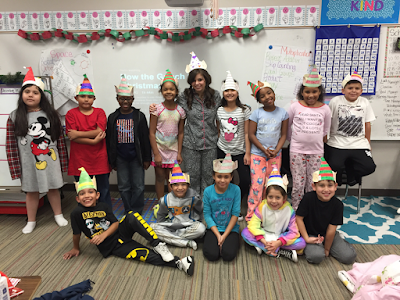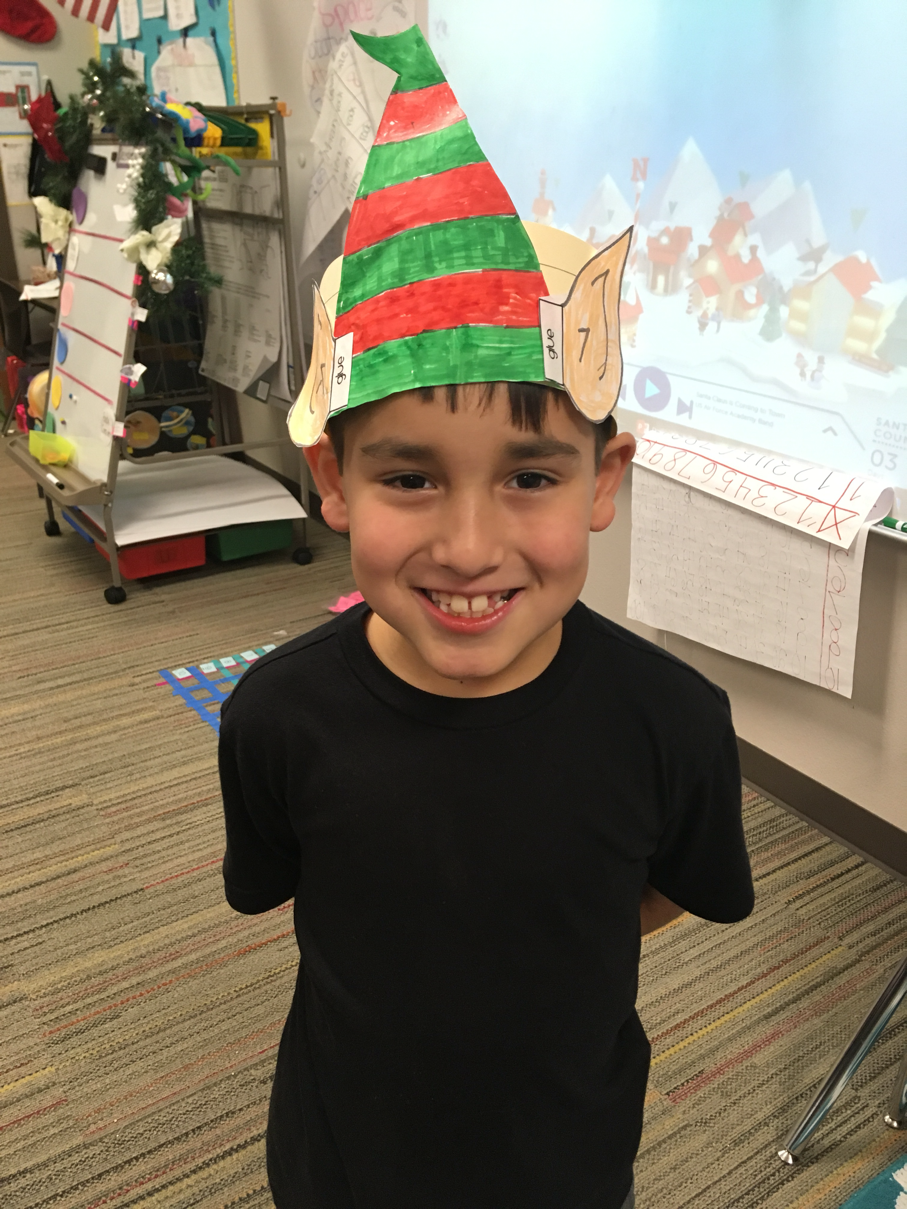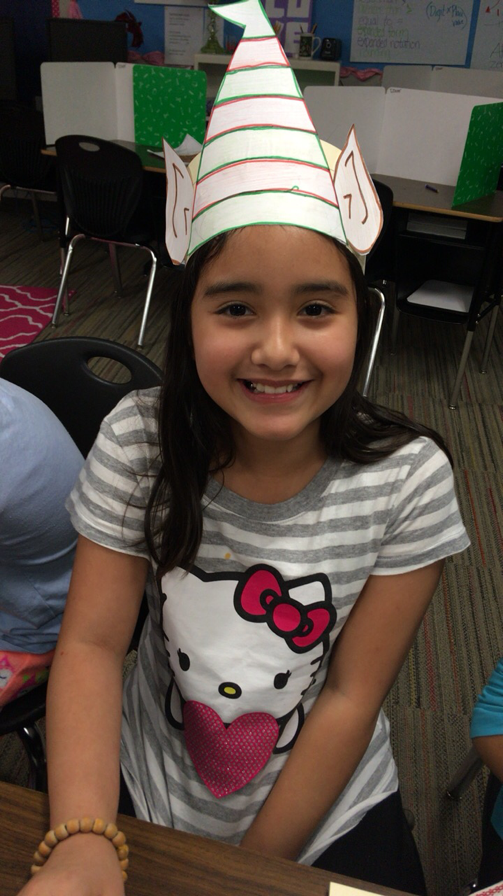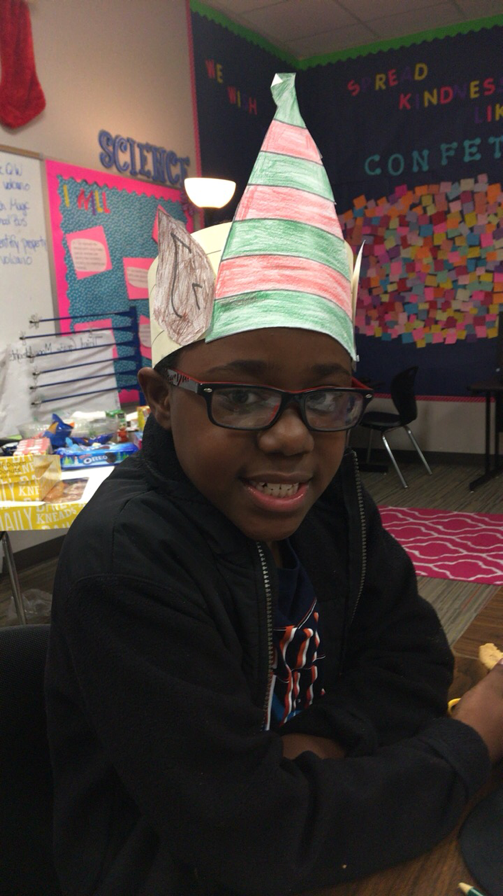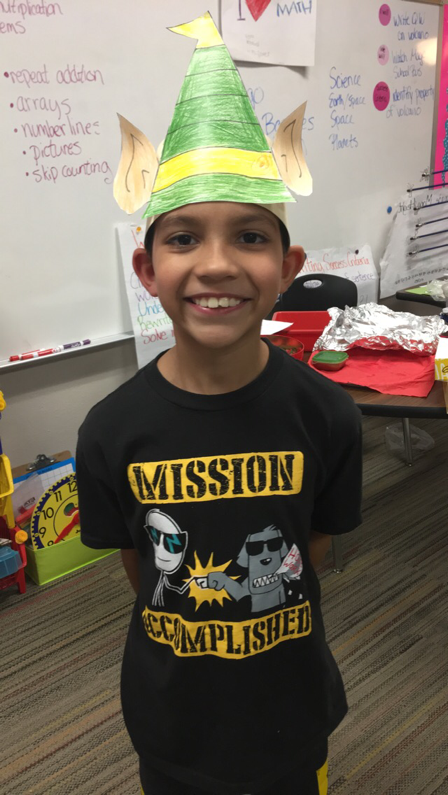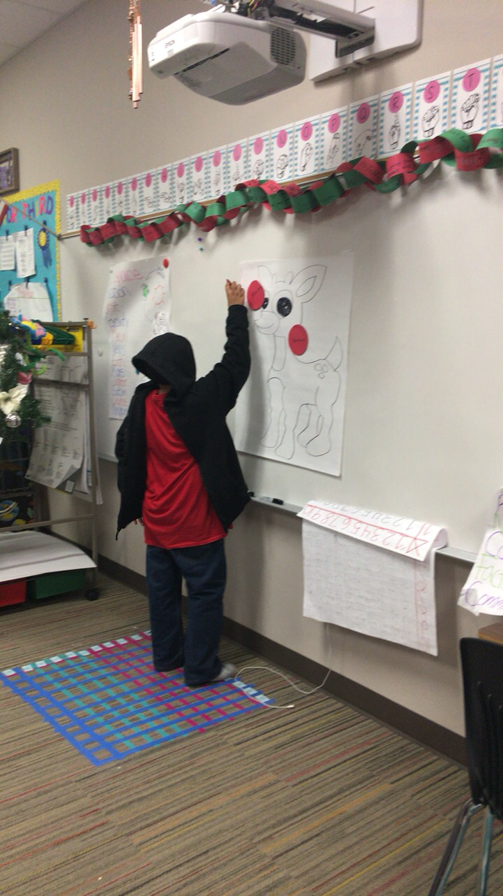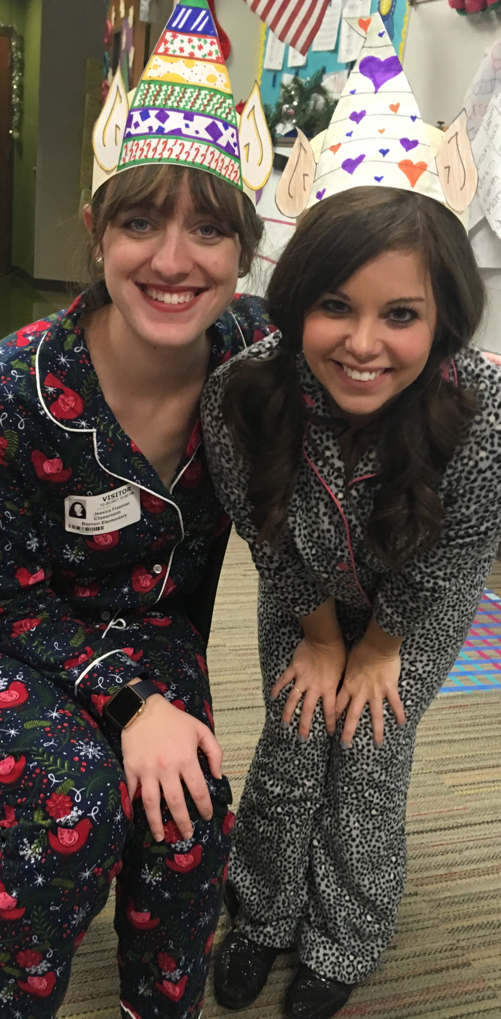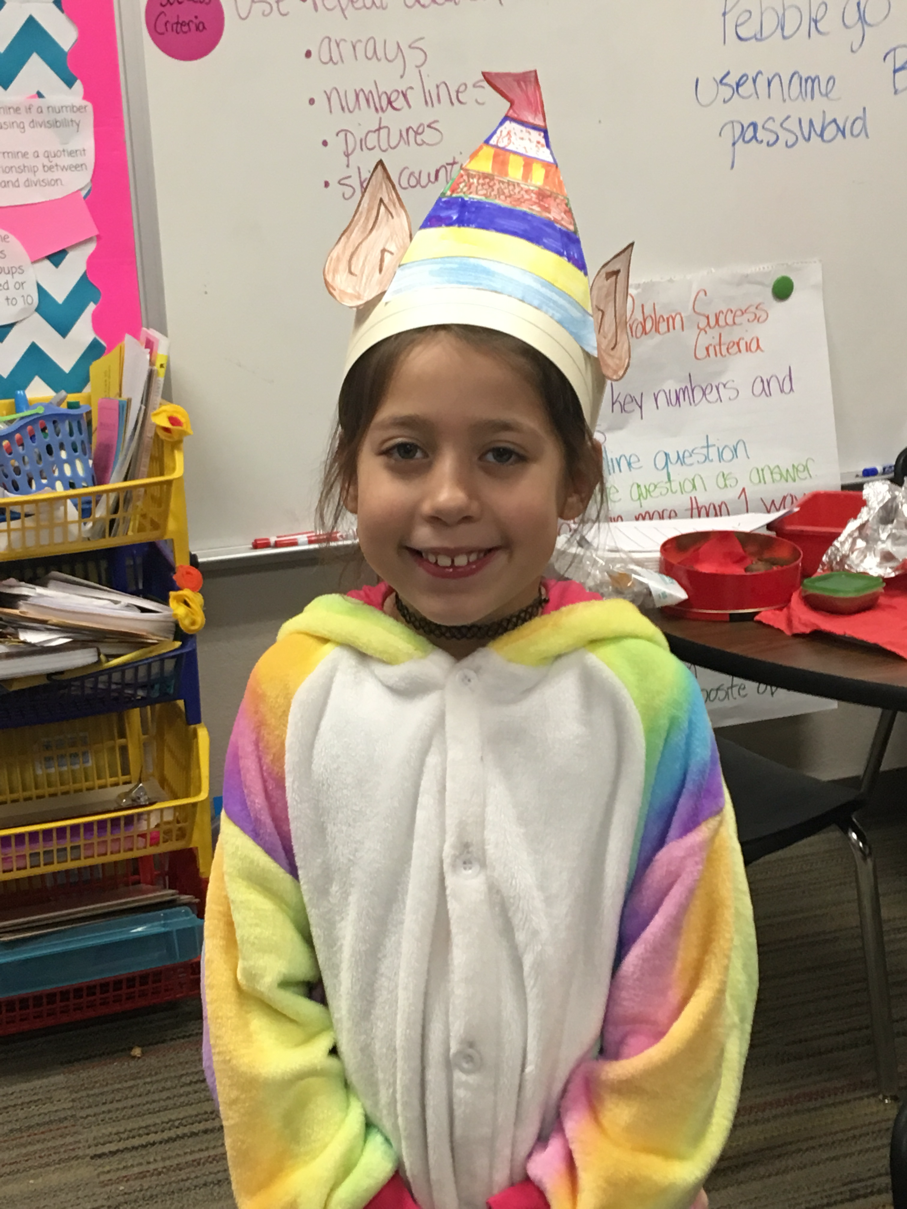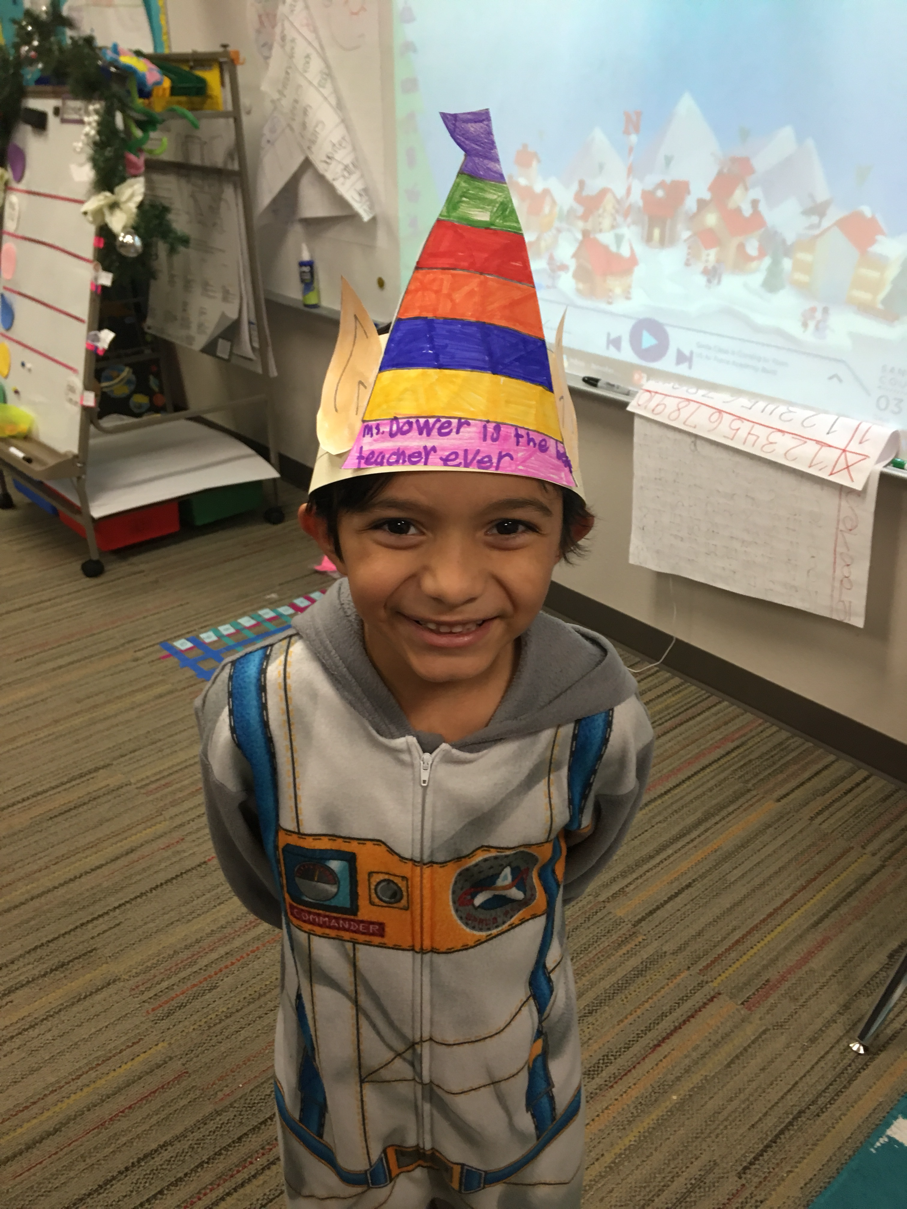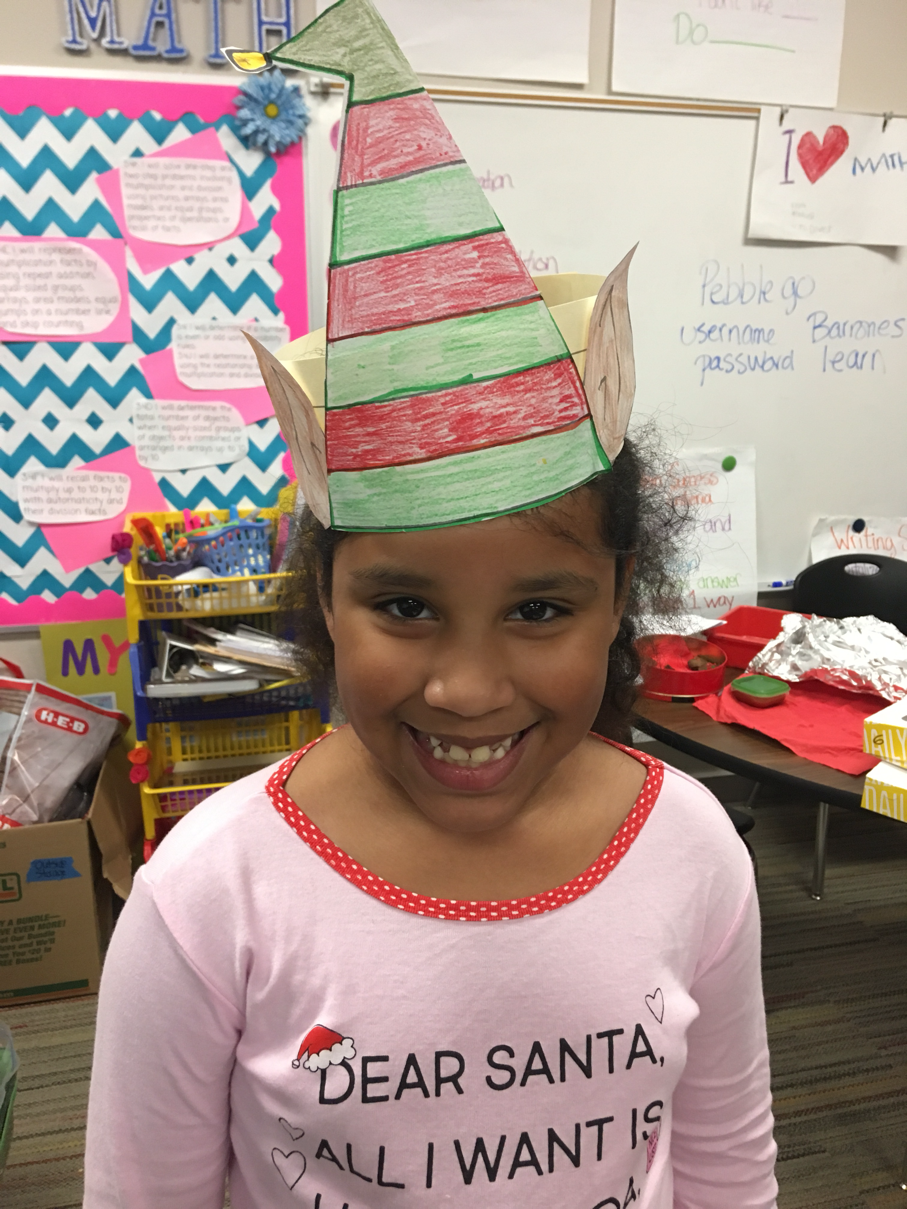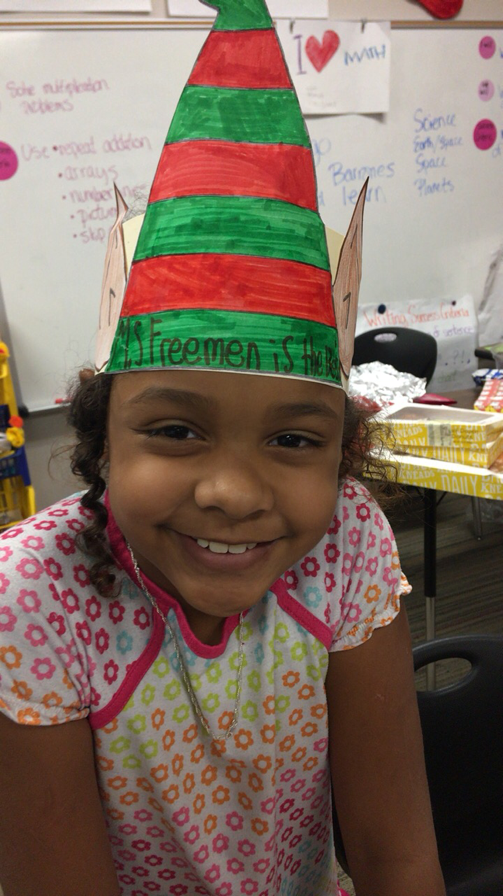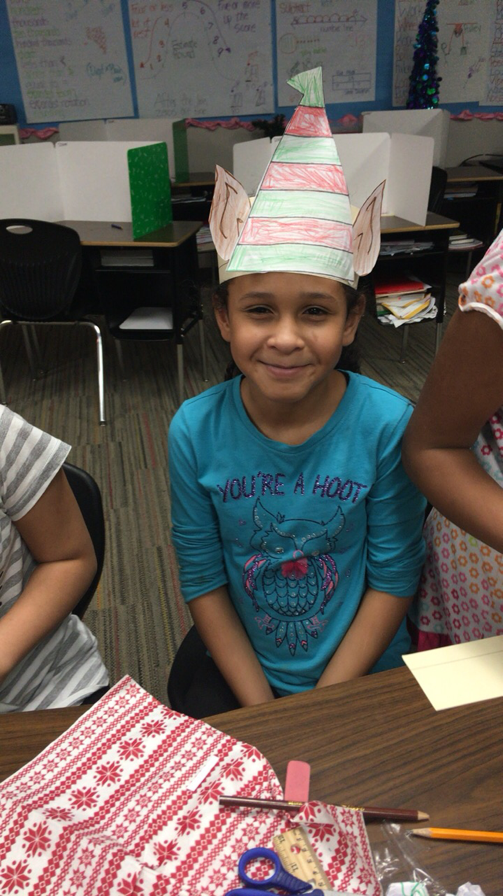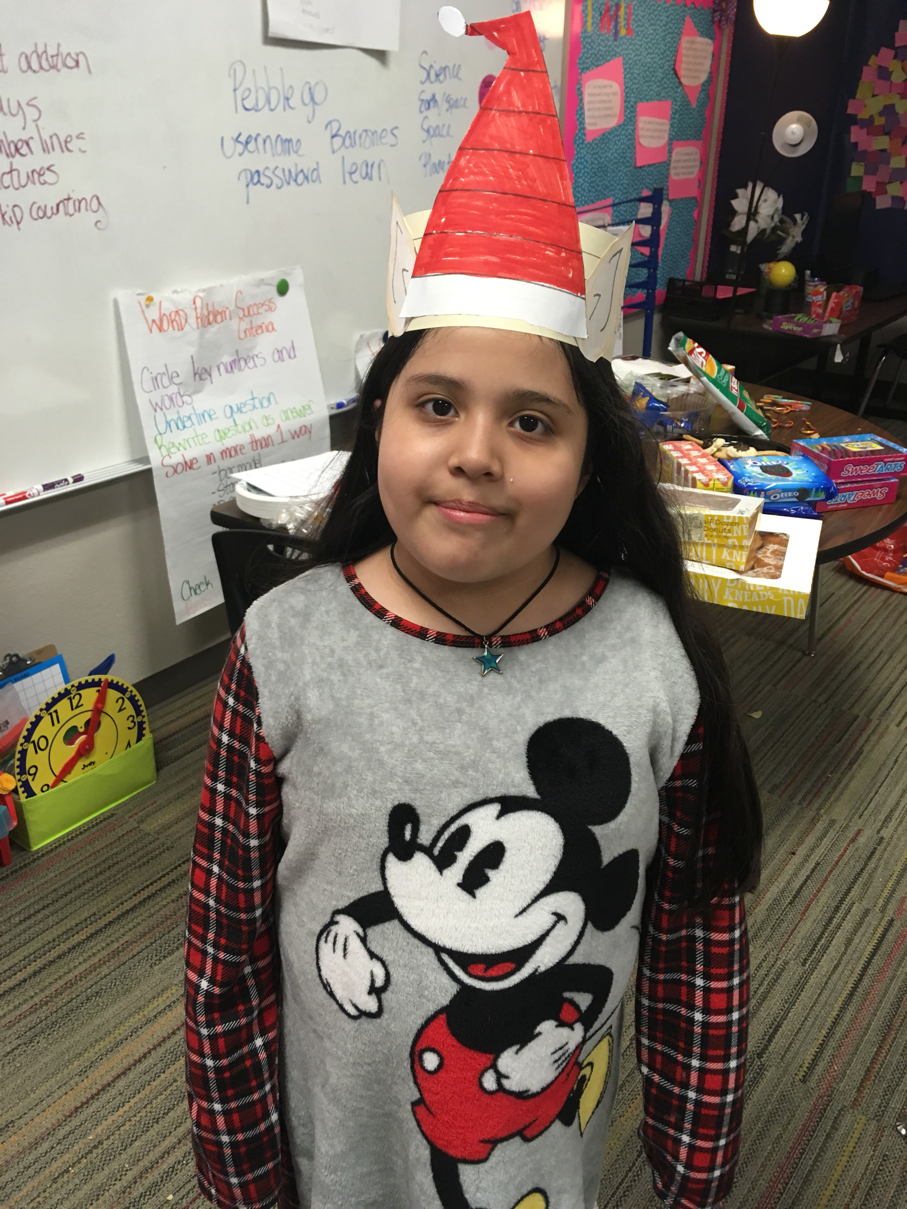Tuesday, February 6, 2018

Unit 6

3.6C I will determine the area of rectangles with whole number side lengths in problems using multiplication.

3.6D I will decompose composite figures  to determine the area.

3.6E I will decompose two congruent two-dimensional figures into parts with equal areas and express the area of each part as a unit fraction of the whole and recognize that equal shares of identical wholes need not have the same shape.

3.6B I will use attributes to recognize
• rhombuses,
• parallelograms,
• trapezoids,
• rectangles, and
• squares
and draw examples of quadrilaterals that do not belong to any of these subcategories.

3.6A I will classify and sort two- and three-dimensional figures, including
• cones,
• cylinders,
• spheres,
• triangular prisms,
• rectangular prisms,
• cubes.

Monday, January 29, 2018

Standard Algorithm

Today we're moving away from partial products and decomposing and we're learning the standard algorithm for 2 digit multiplication!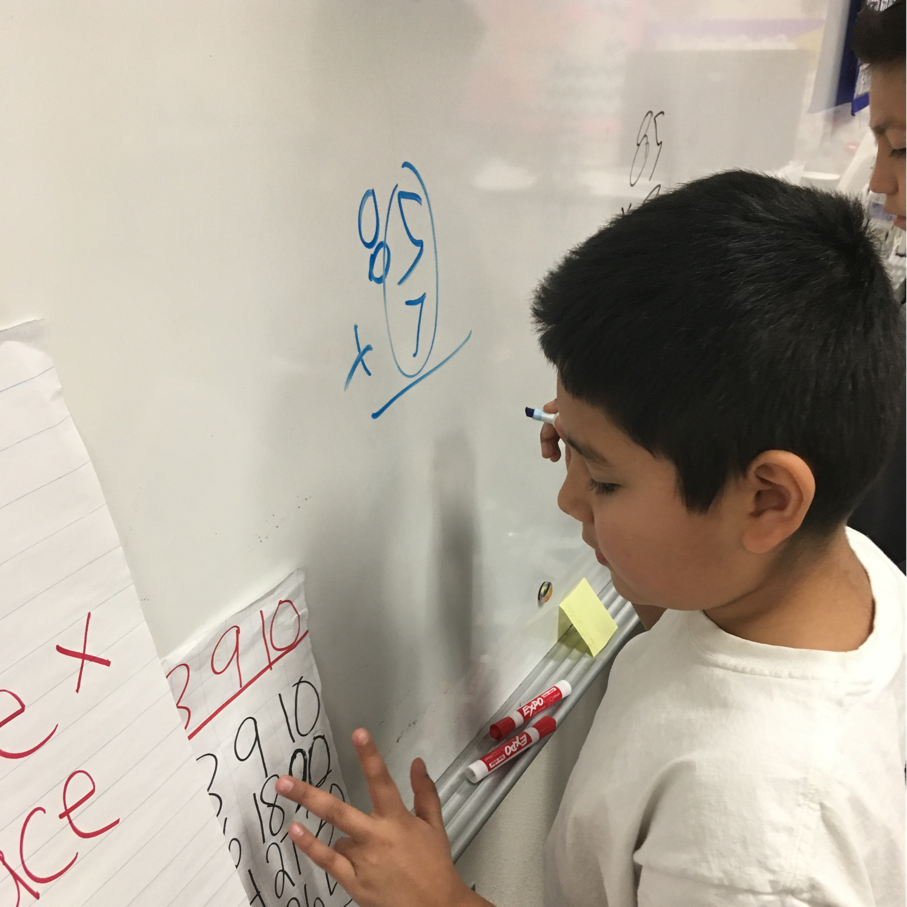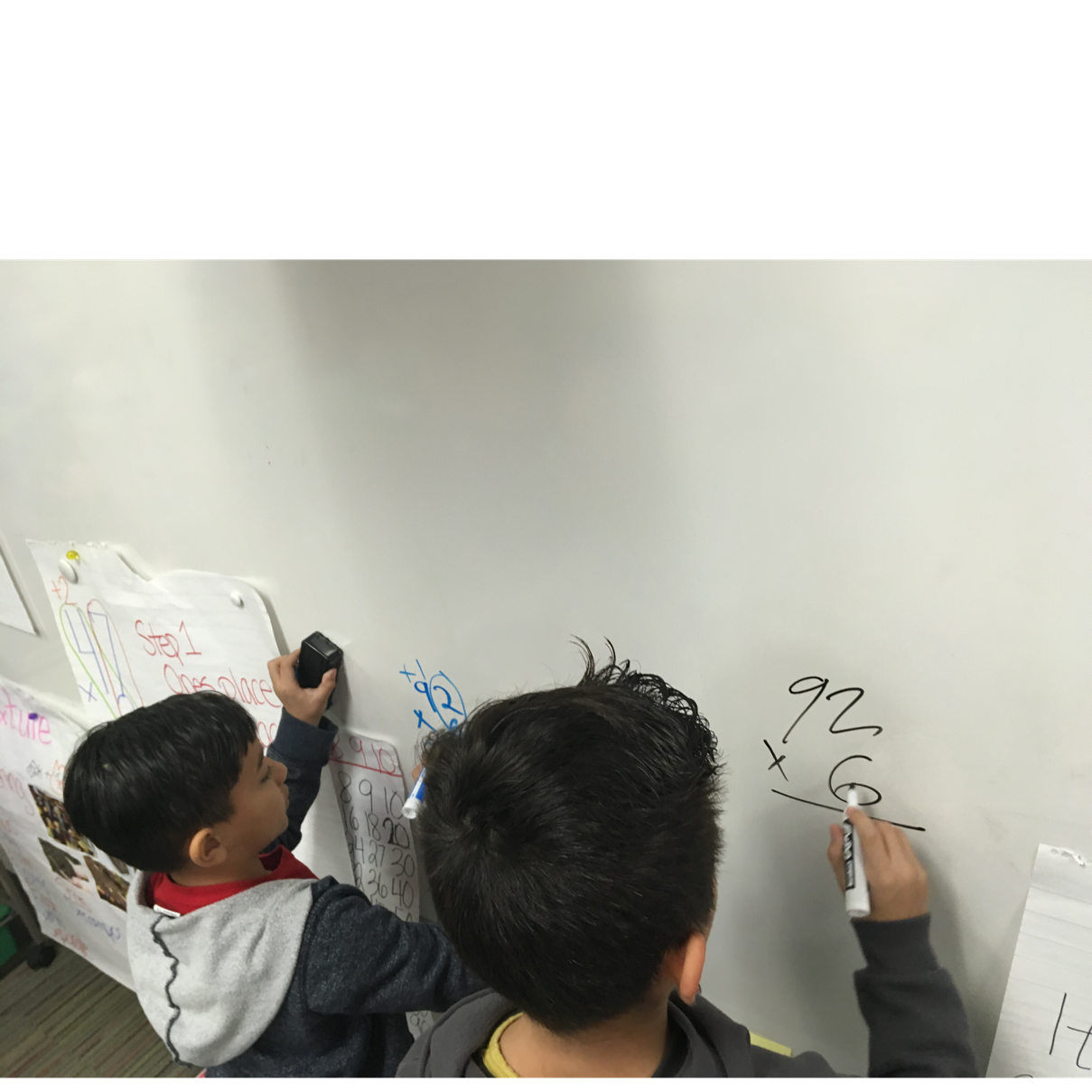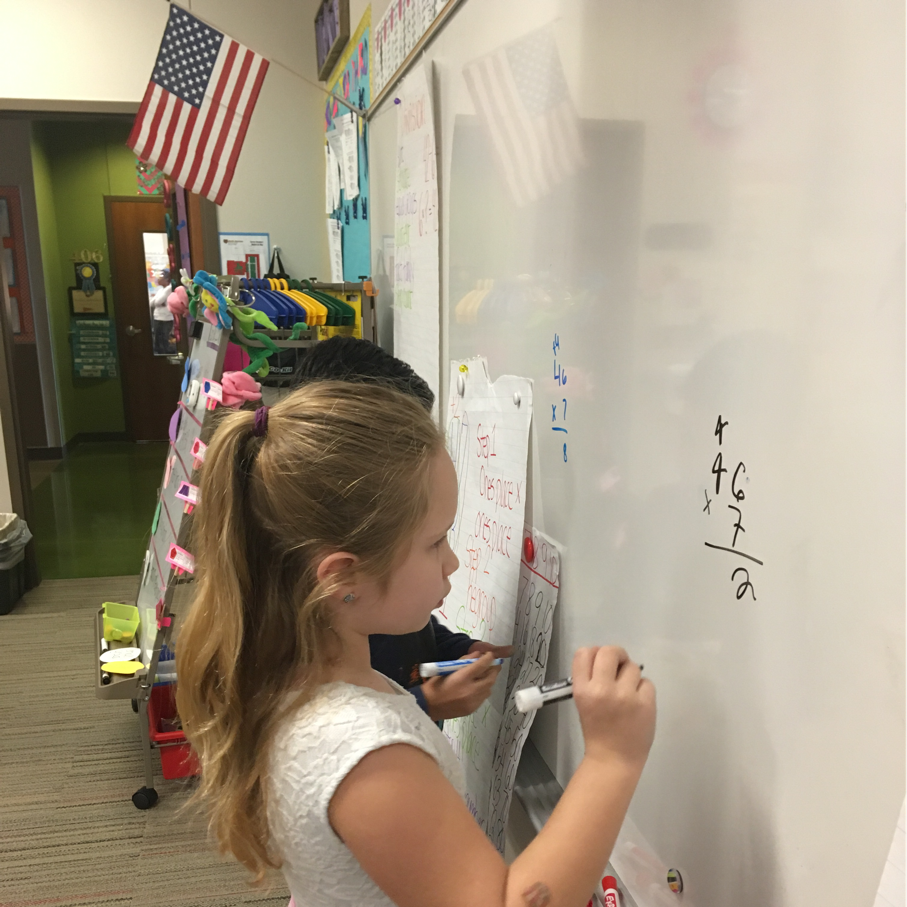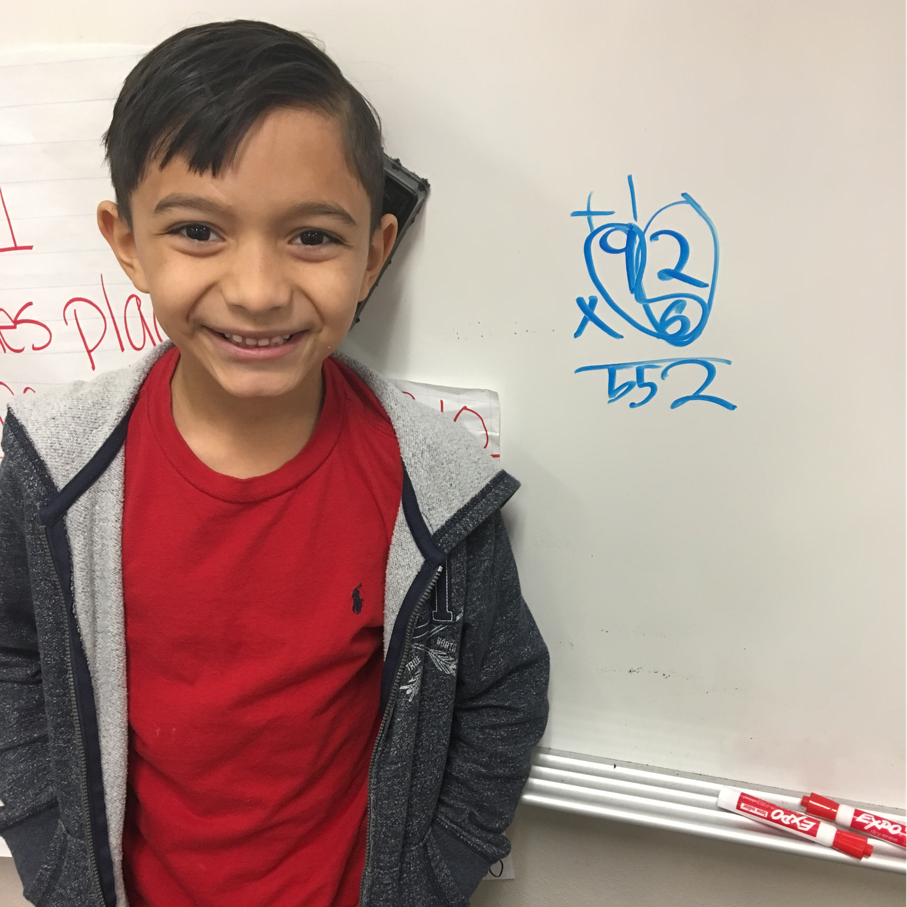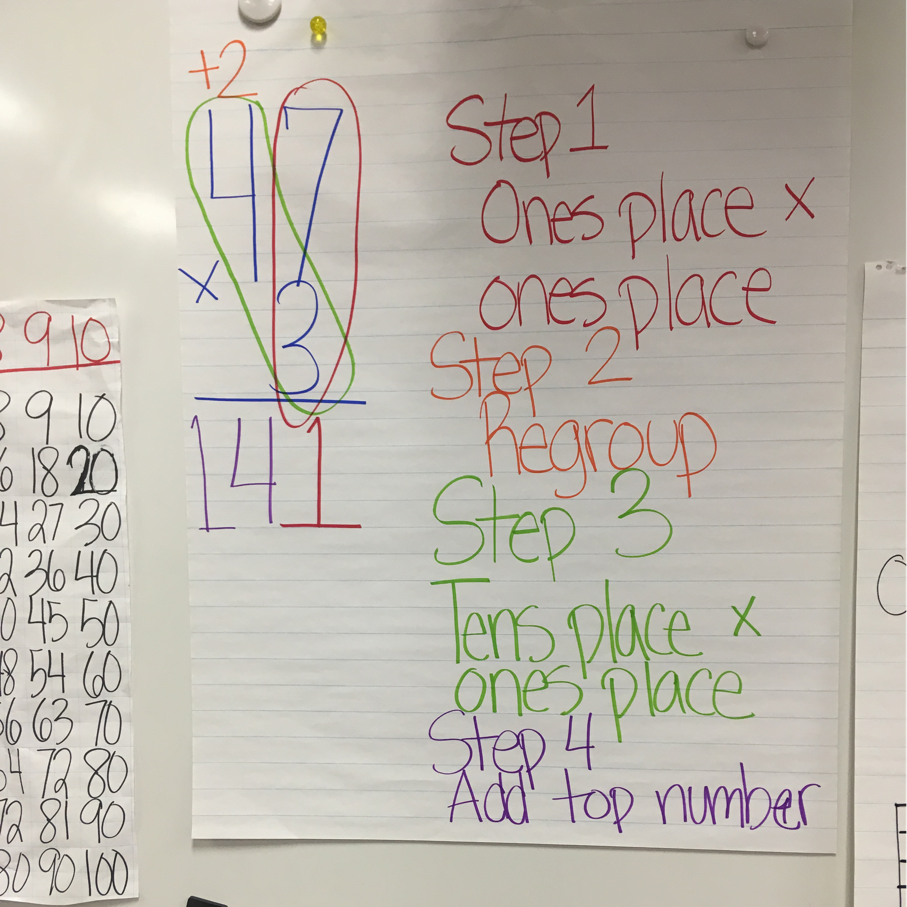Monday, January 22, 2018

Soil

Today Dr. Dower is coming to show us some different kinds of SOIL!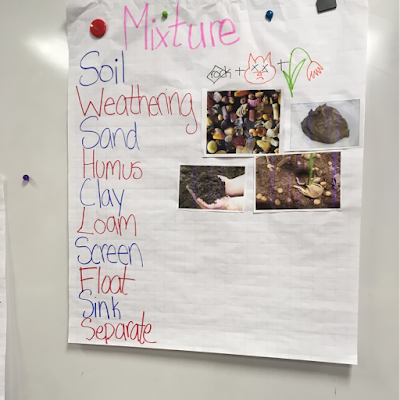First, we reviewed with the kiddos what we already learned.
We know about soil being the correct word for "dirt."
We know about four different kinds of soil:
Sand, Humus, Clay, and Loam.

We know a little bit about weathering, which is how things get broken or worn down.

Today we'll be talking about separating the mixtures and dissolving materials in water.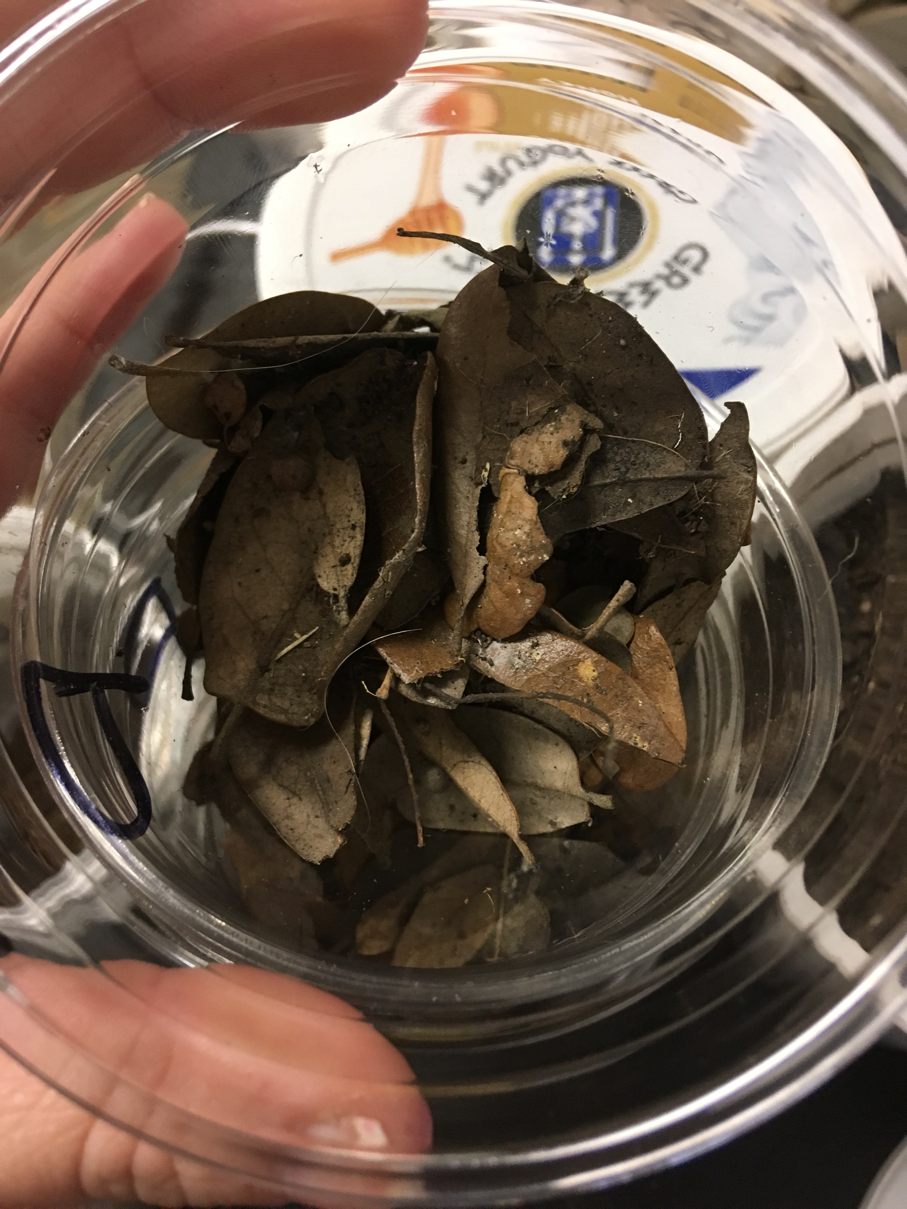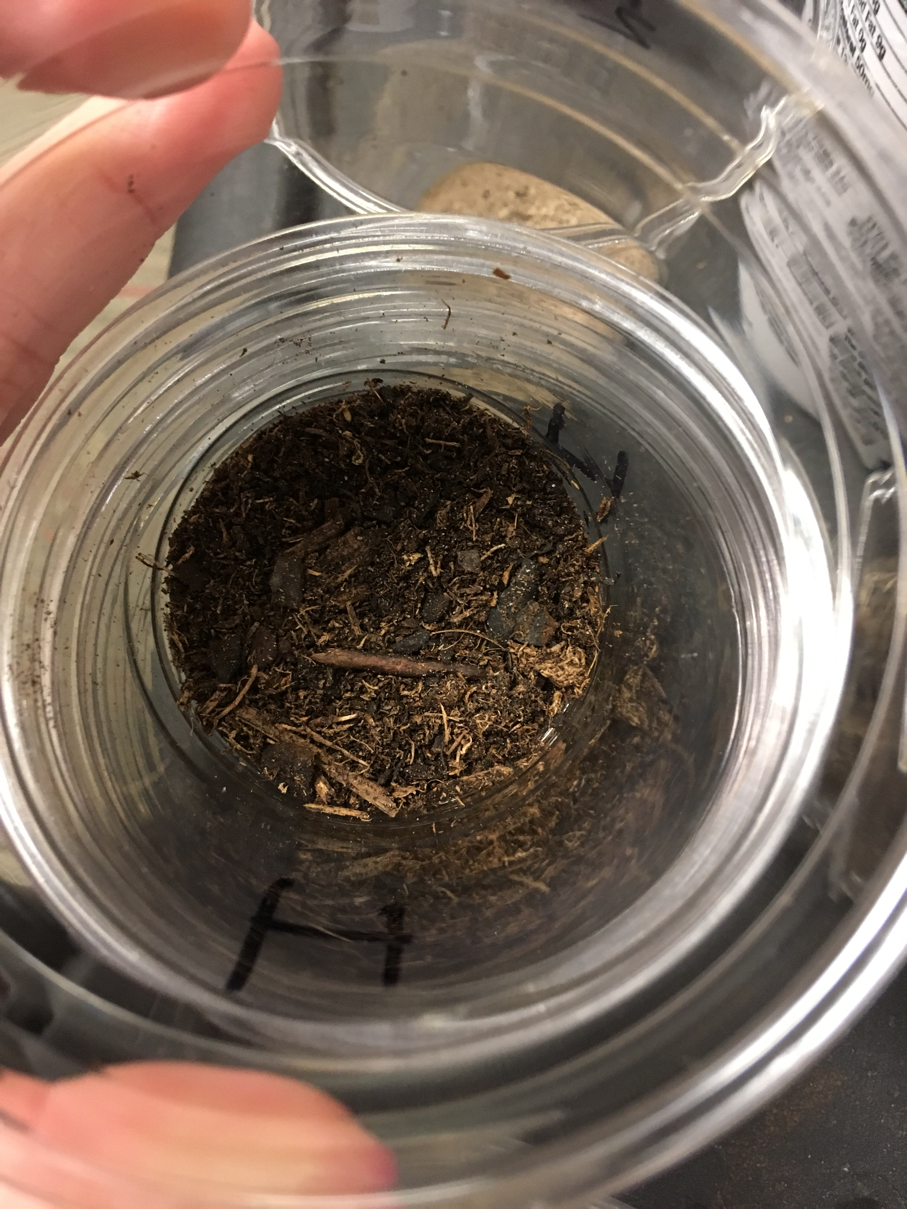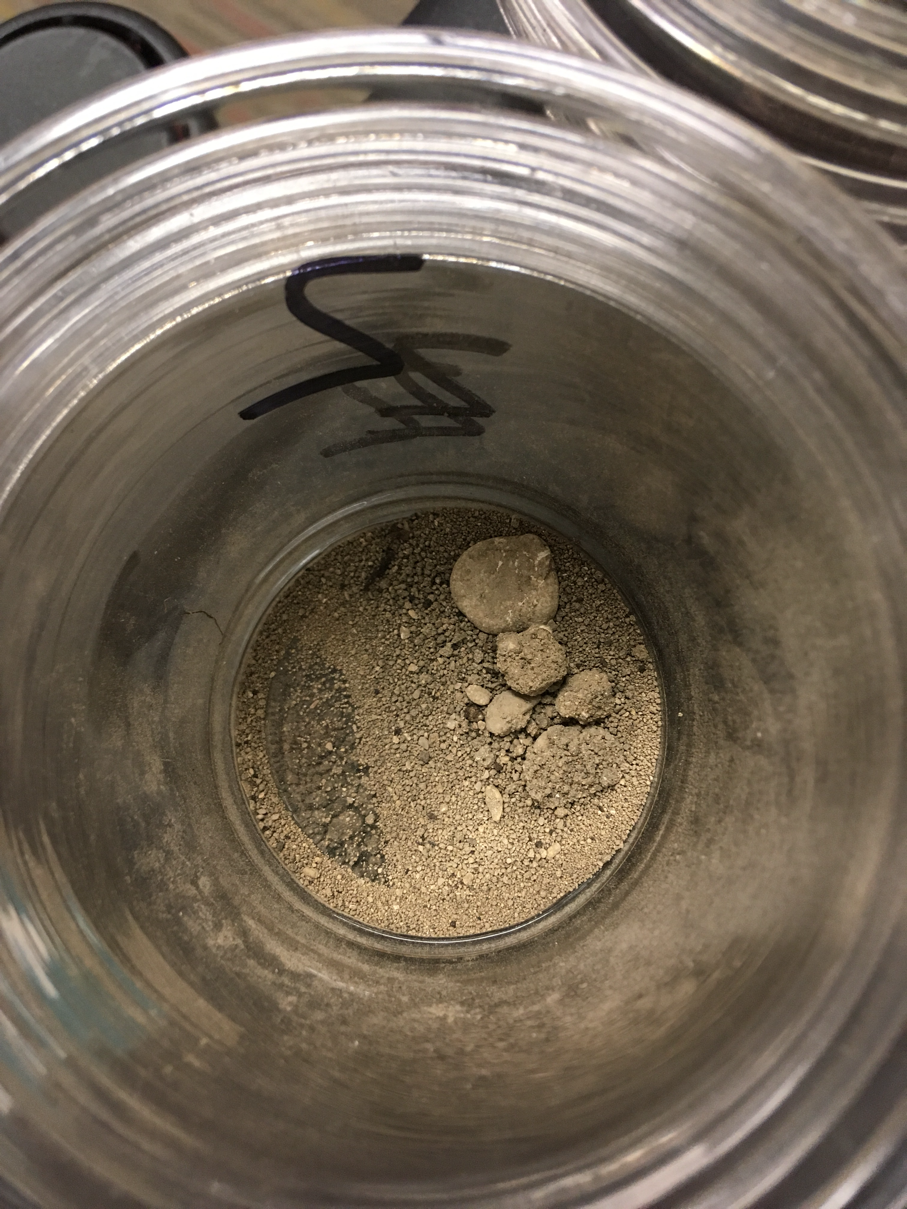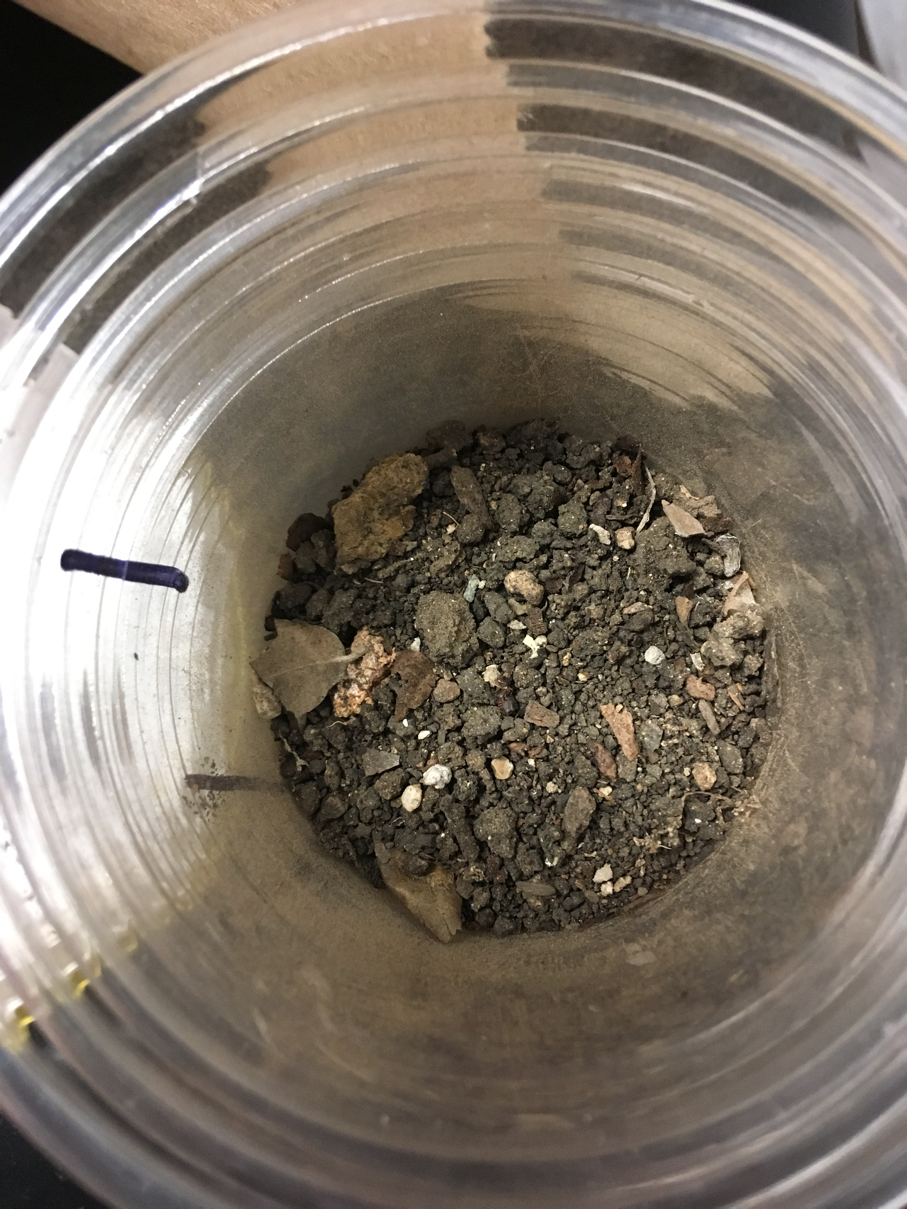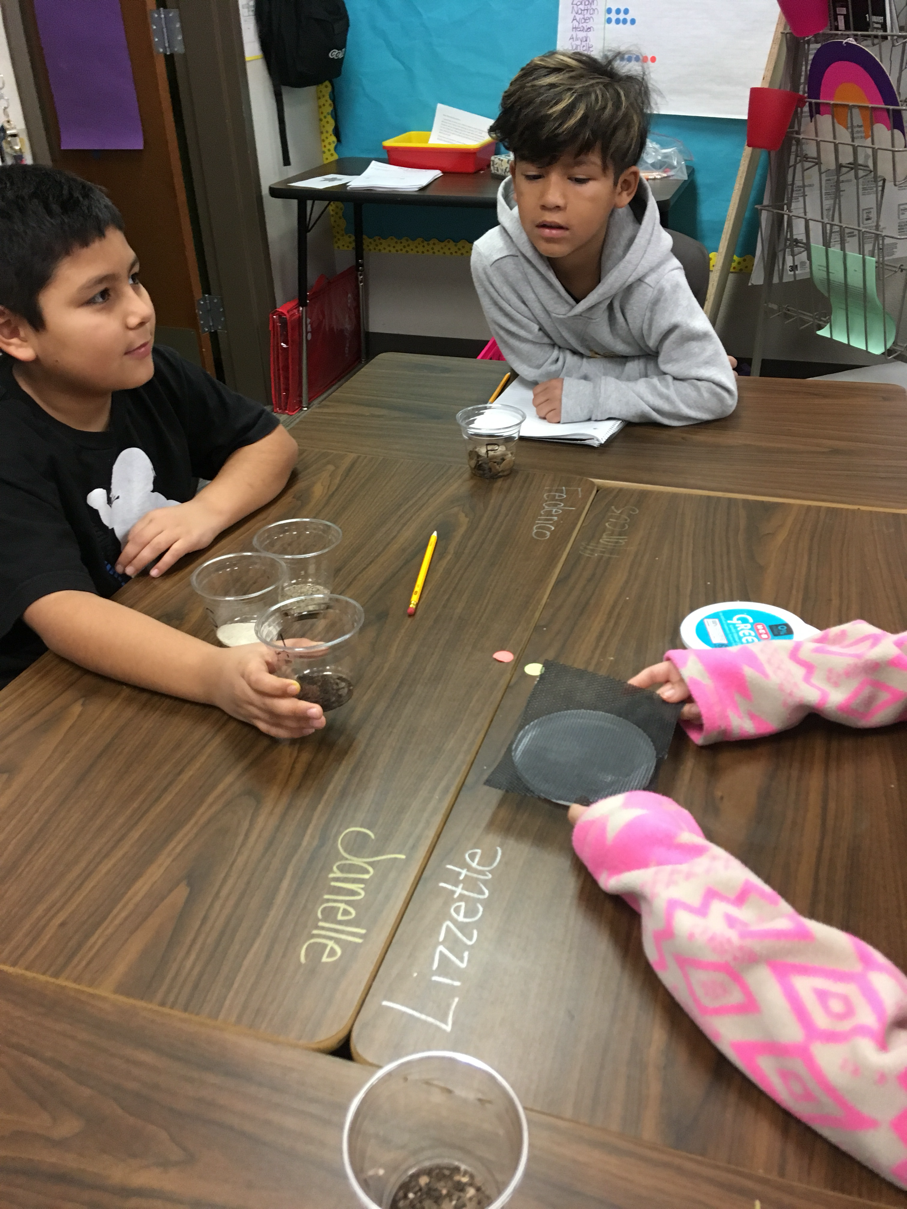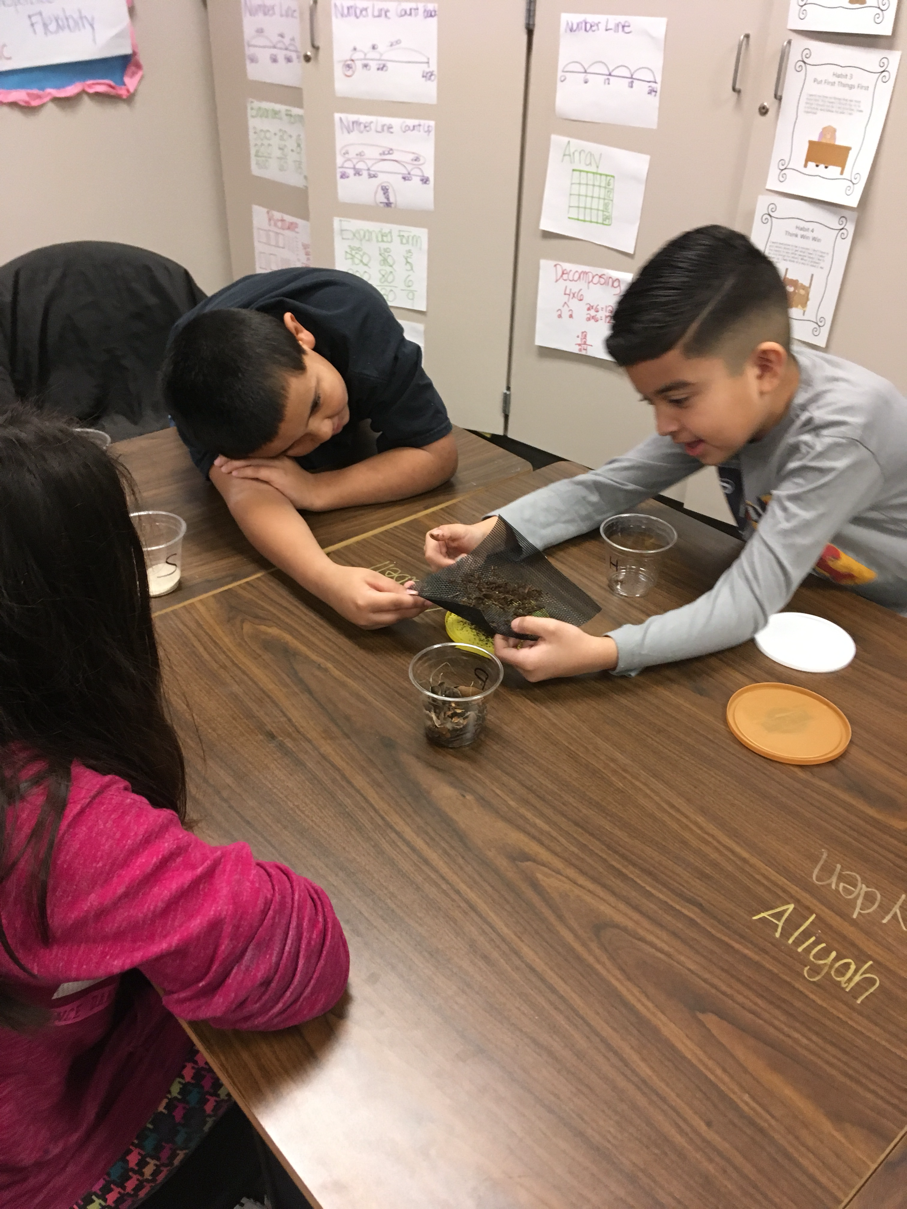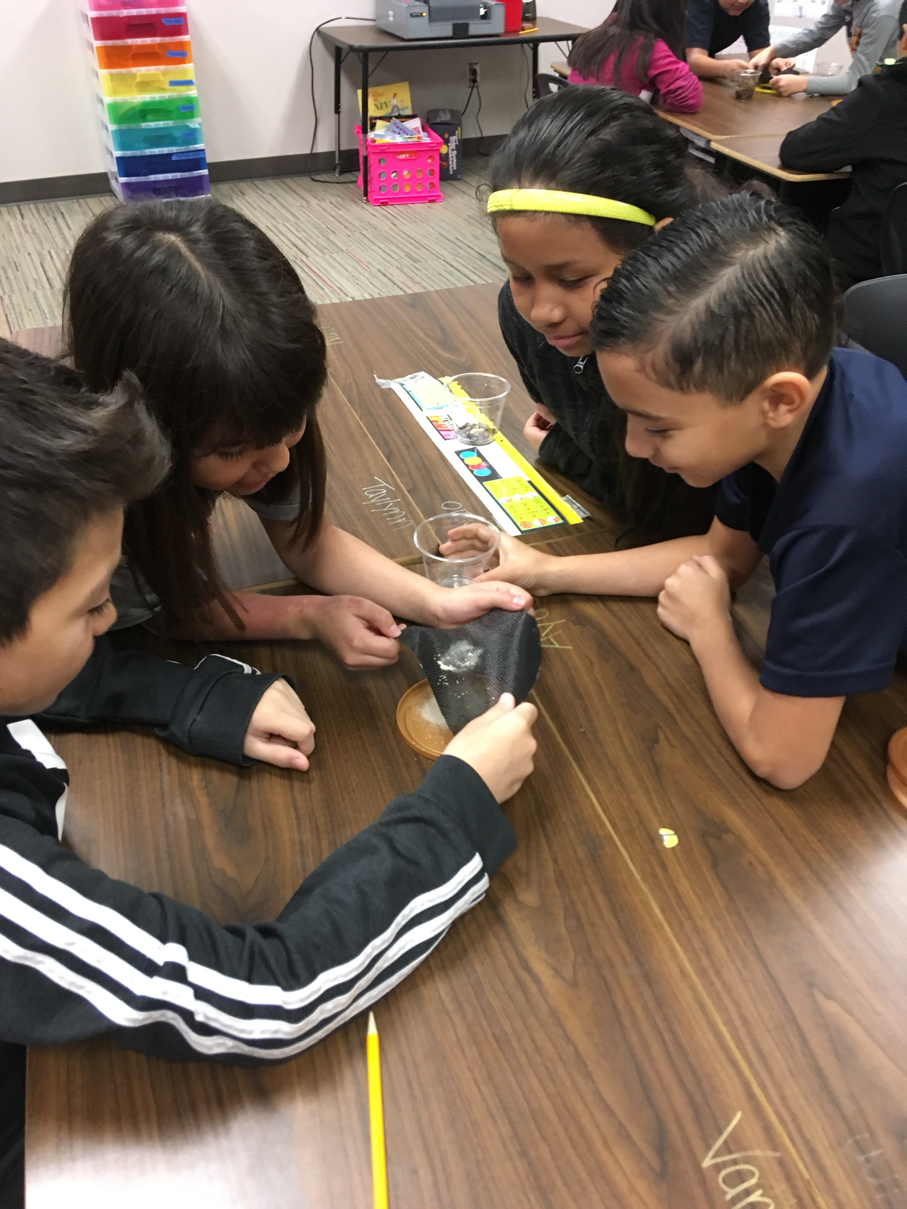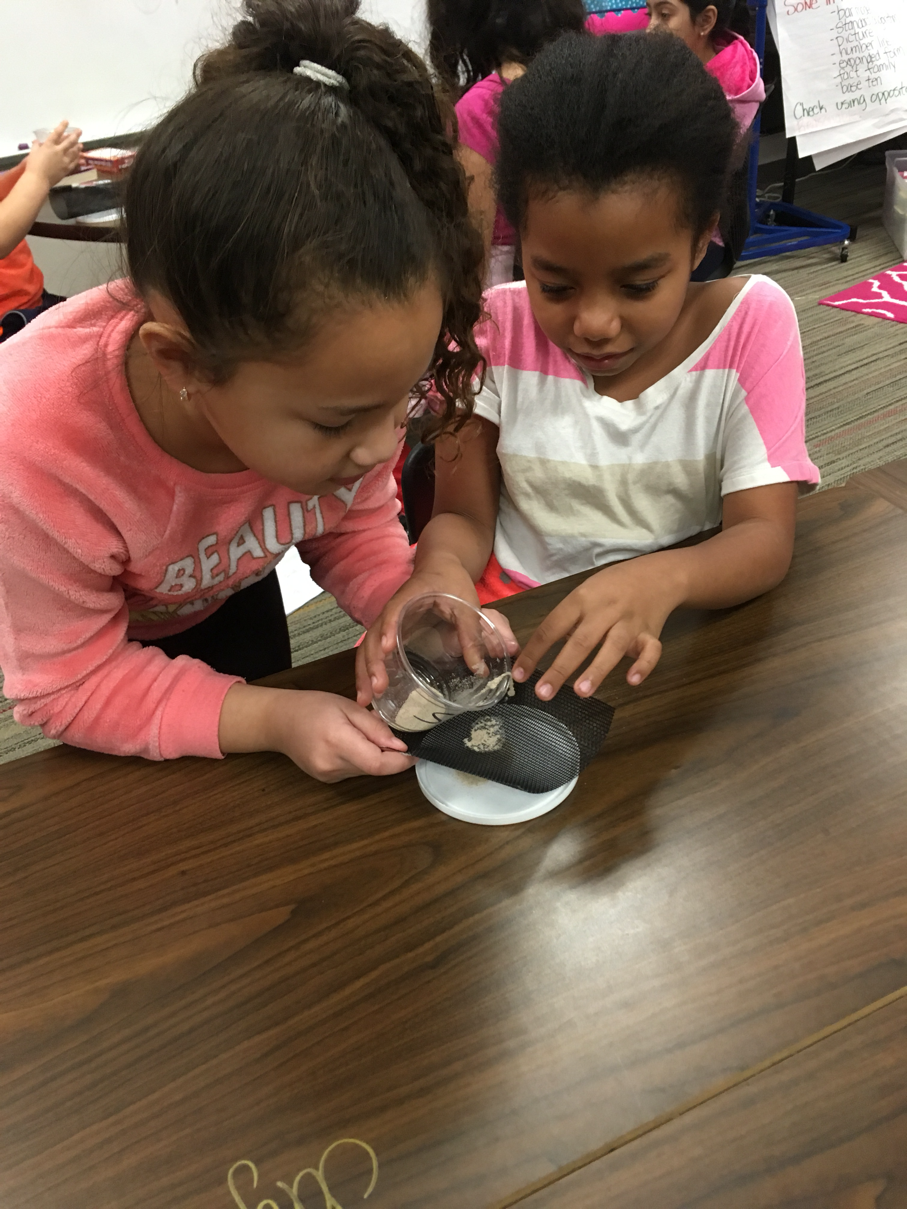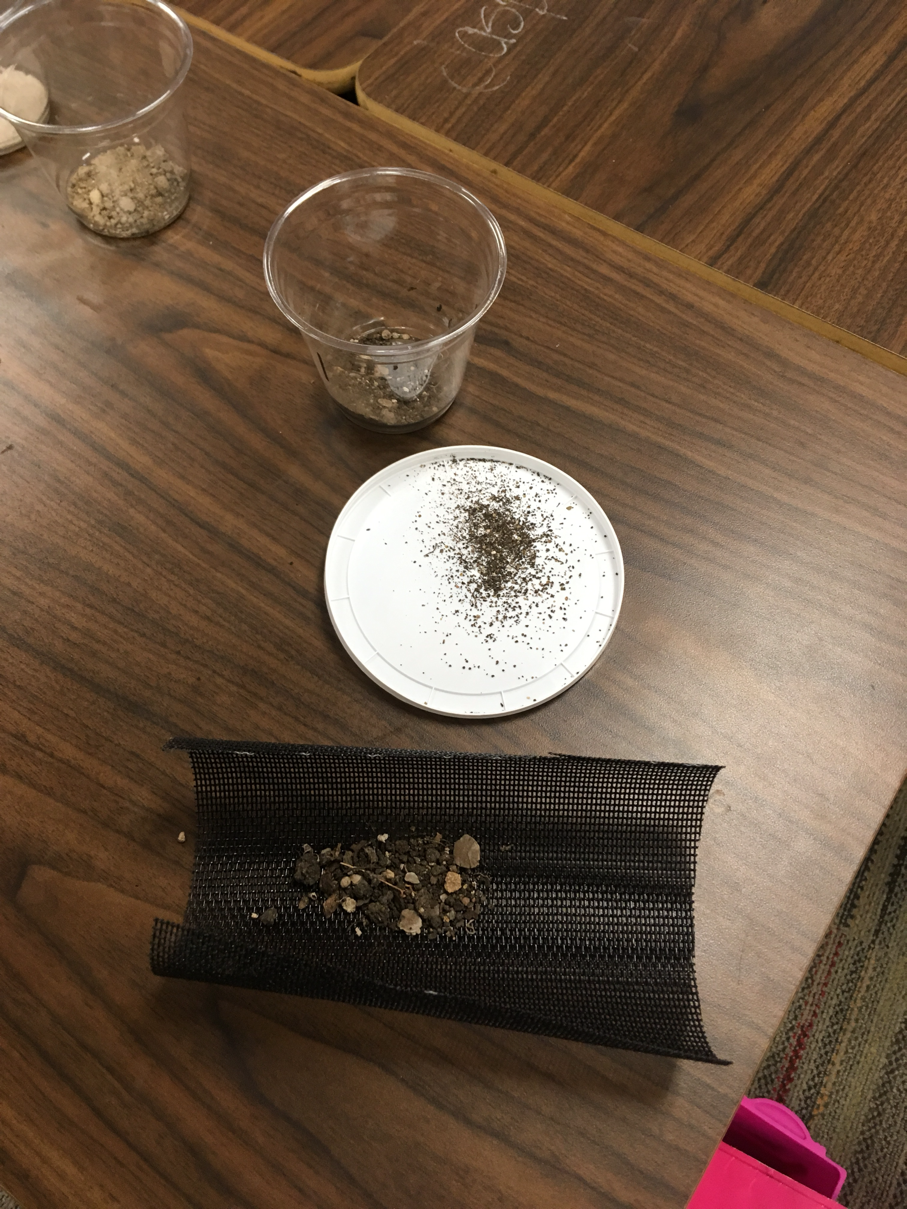After Dr. Dower left to go see another class, we wrote about what happened!
I give them the words I expect them to use, as well as some sentence stems for them to follow.
﻿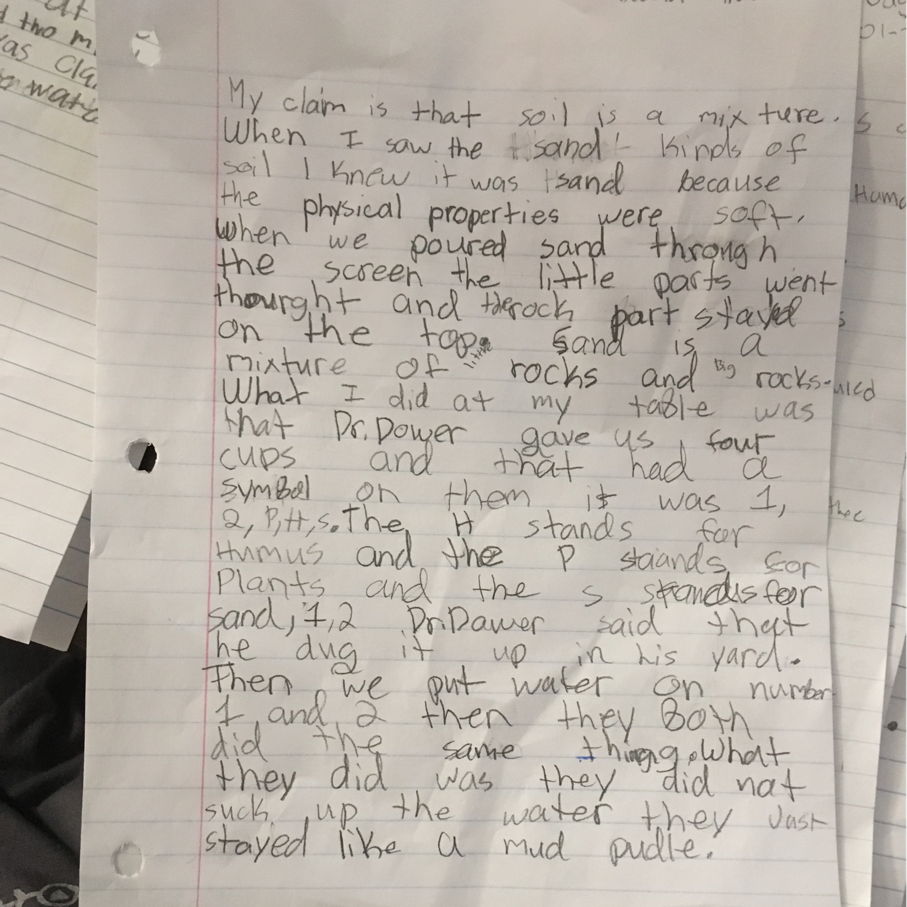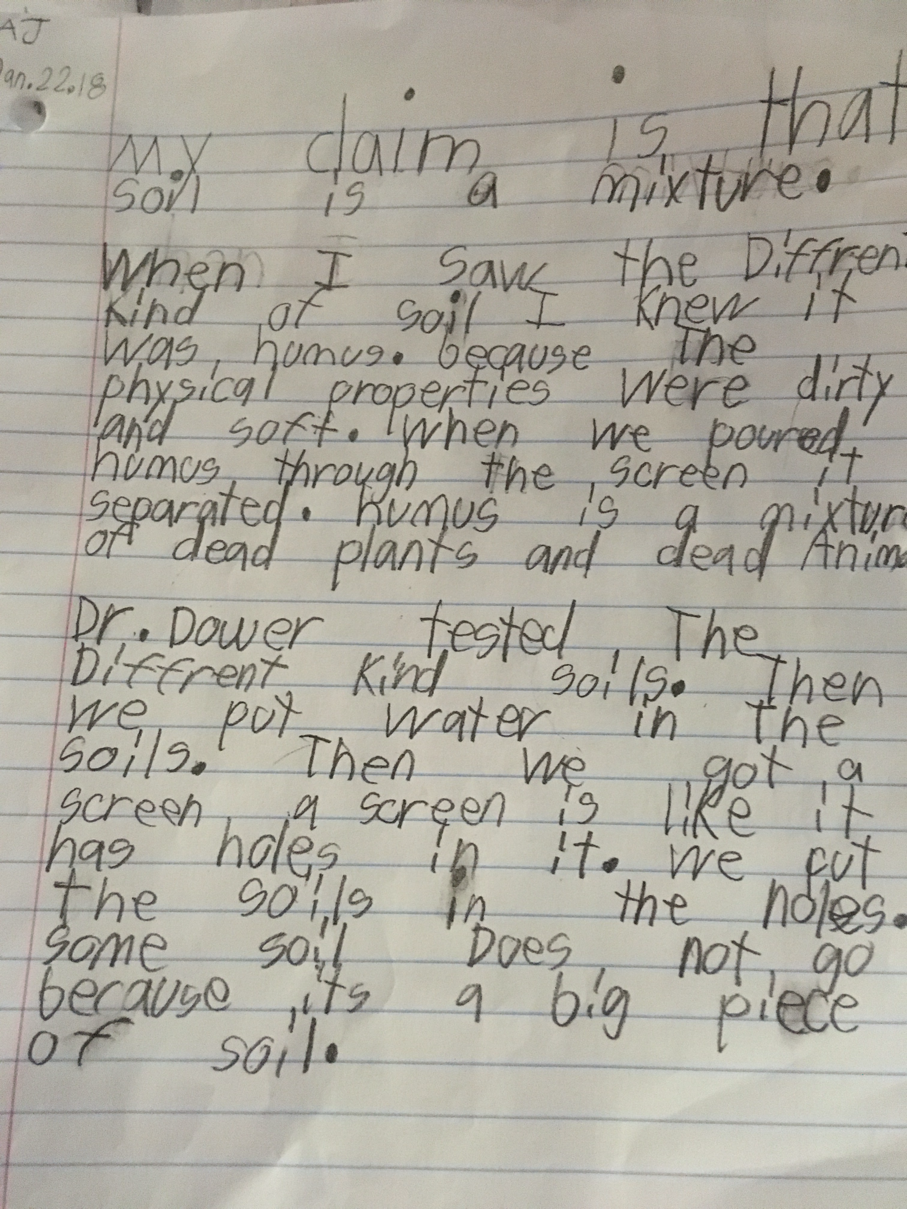Wednesday, January 10, 2018

Decomposing in multiplication

Today we talked about decomposing and reviewed our nines trick.
Our nines trick is with our fingers. We put all ten fingers flat. Then we put down the finger that we're multiplying nine by.
Then we count the fingers to the left of that finger. That's the tens place. The fingers to the right of the down finger is the ones place.
I have Jasper demonstrating in photos below!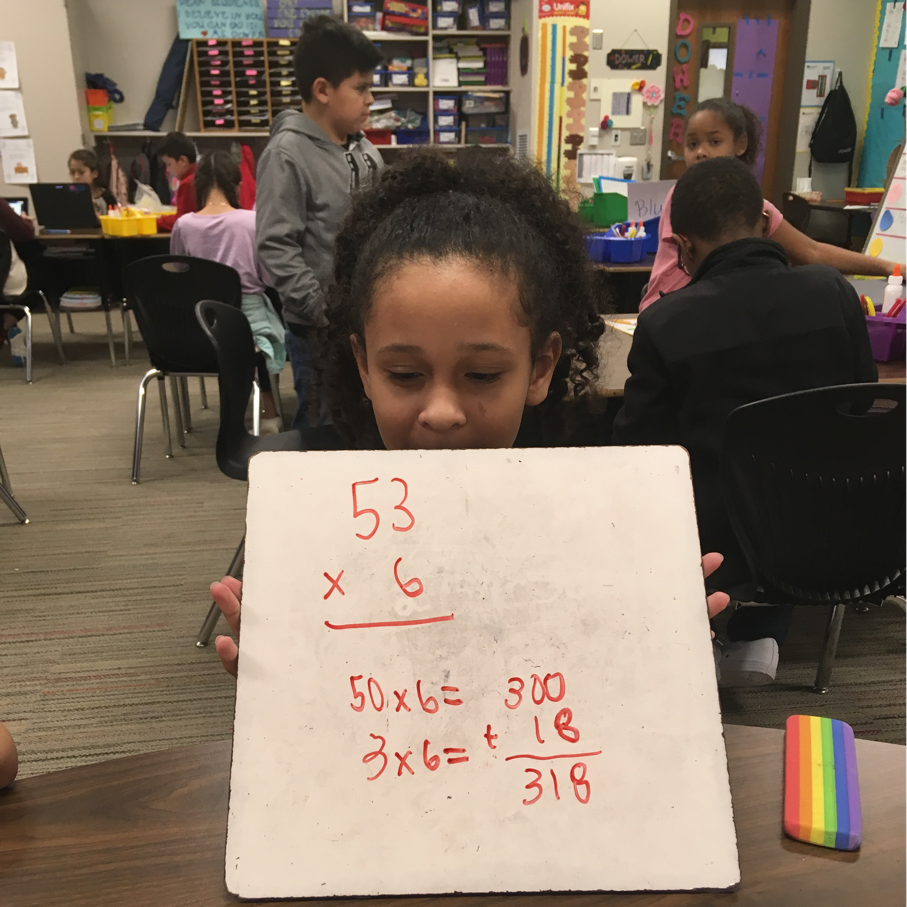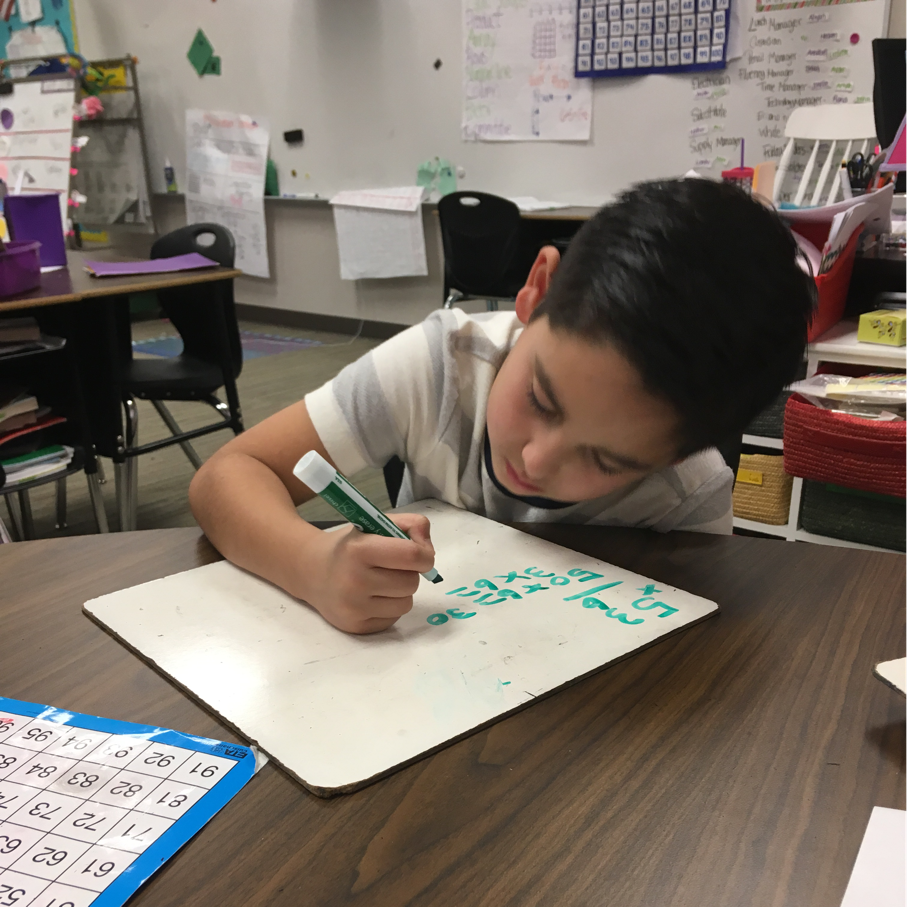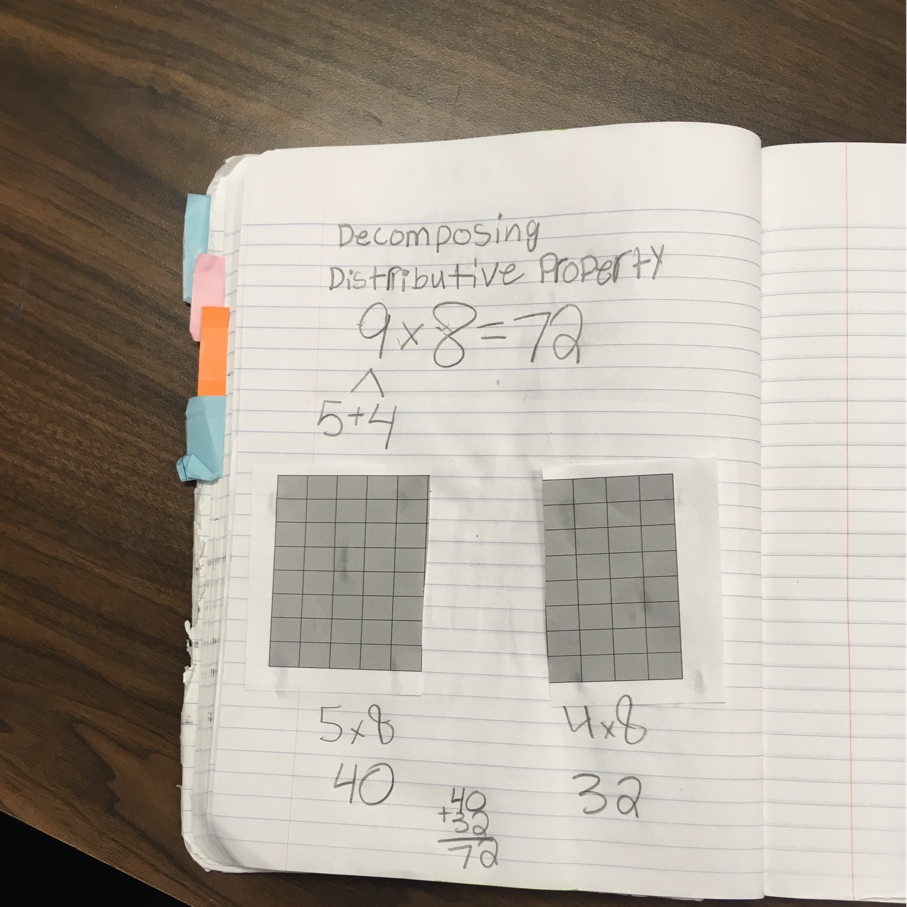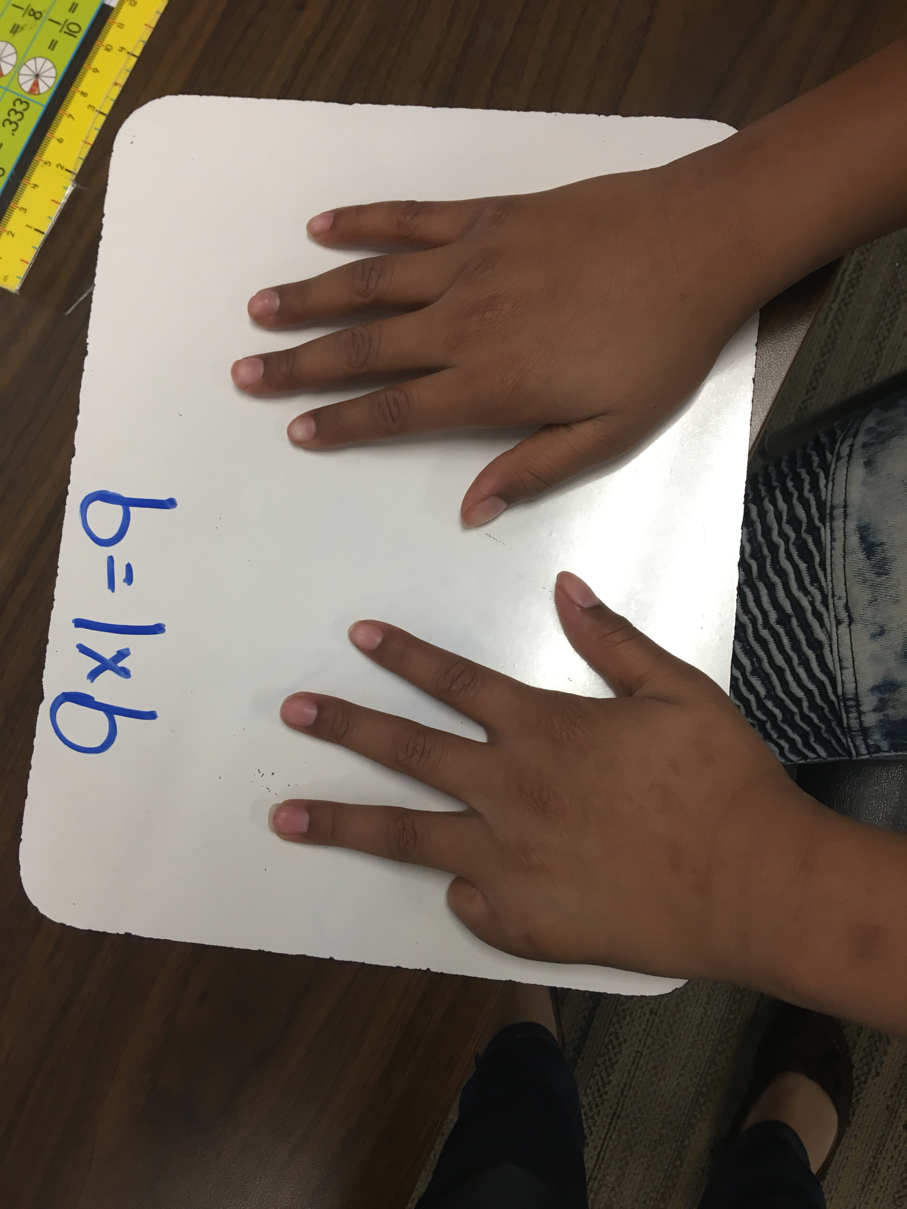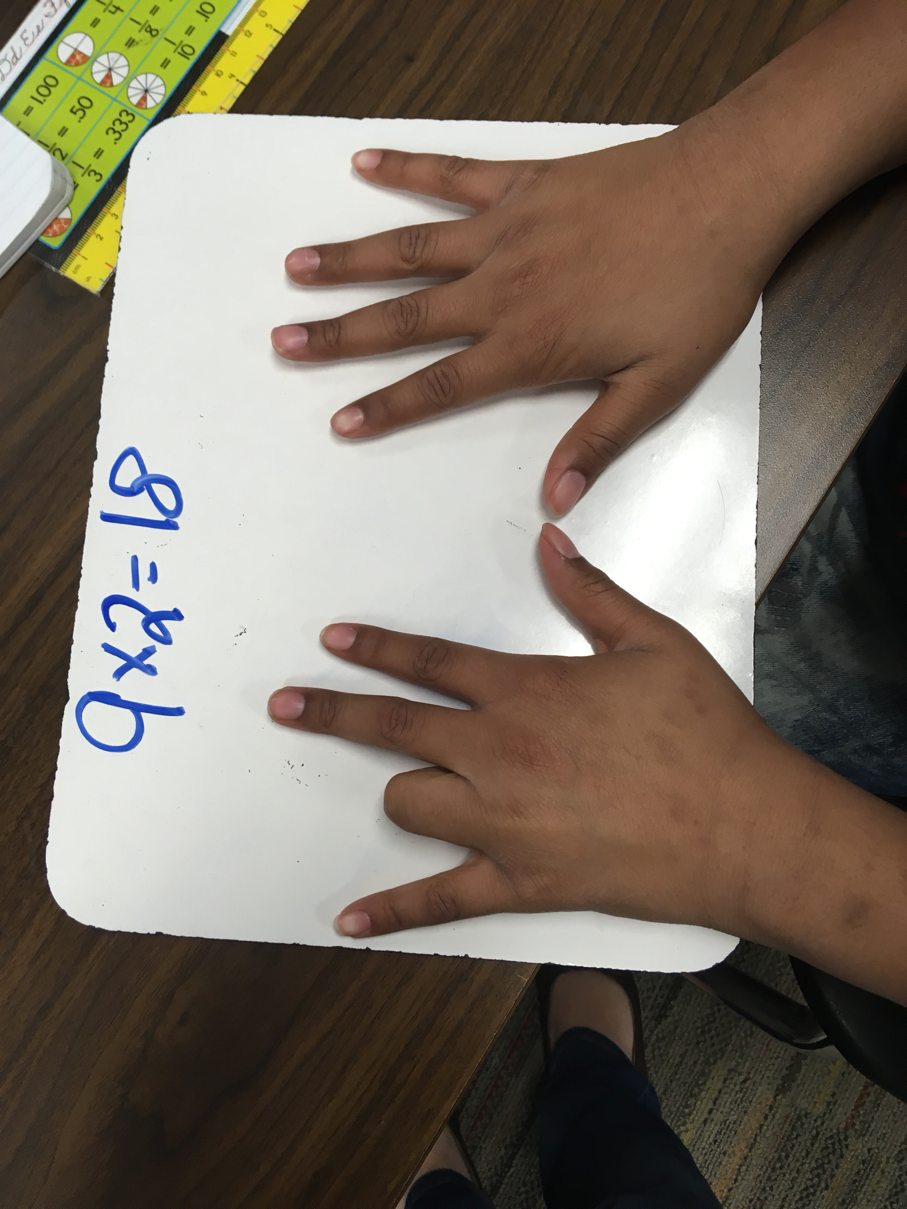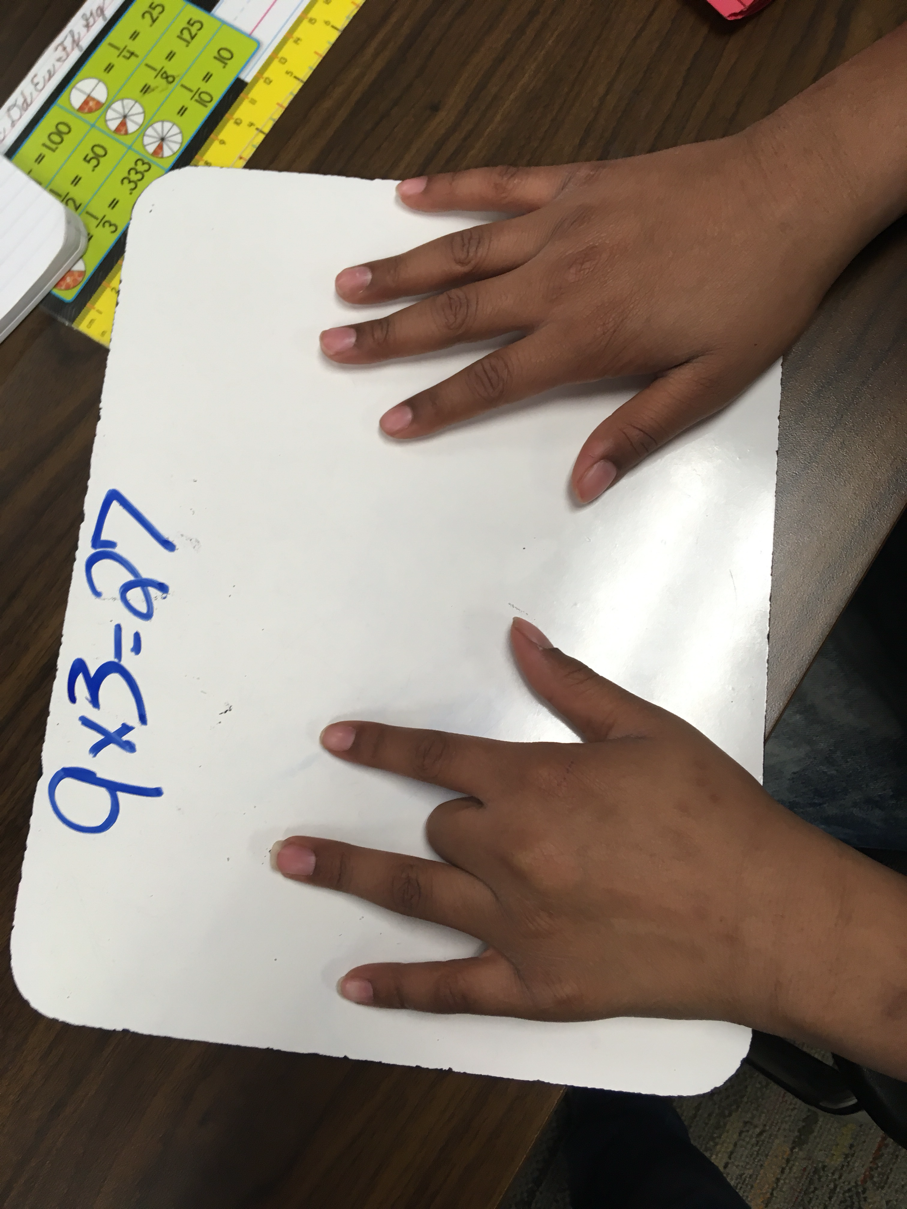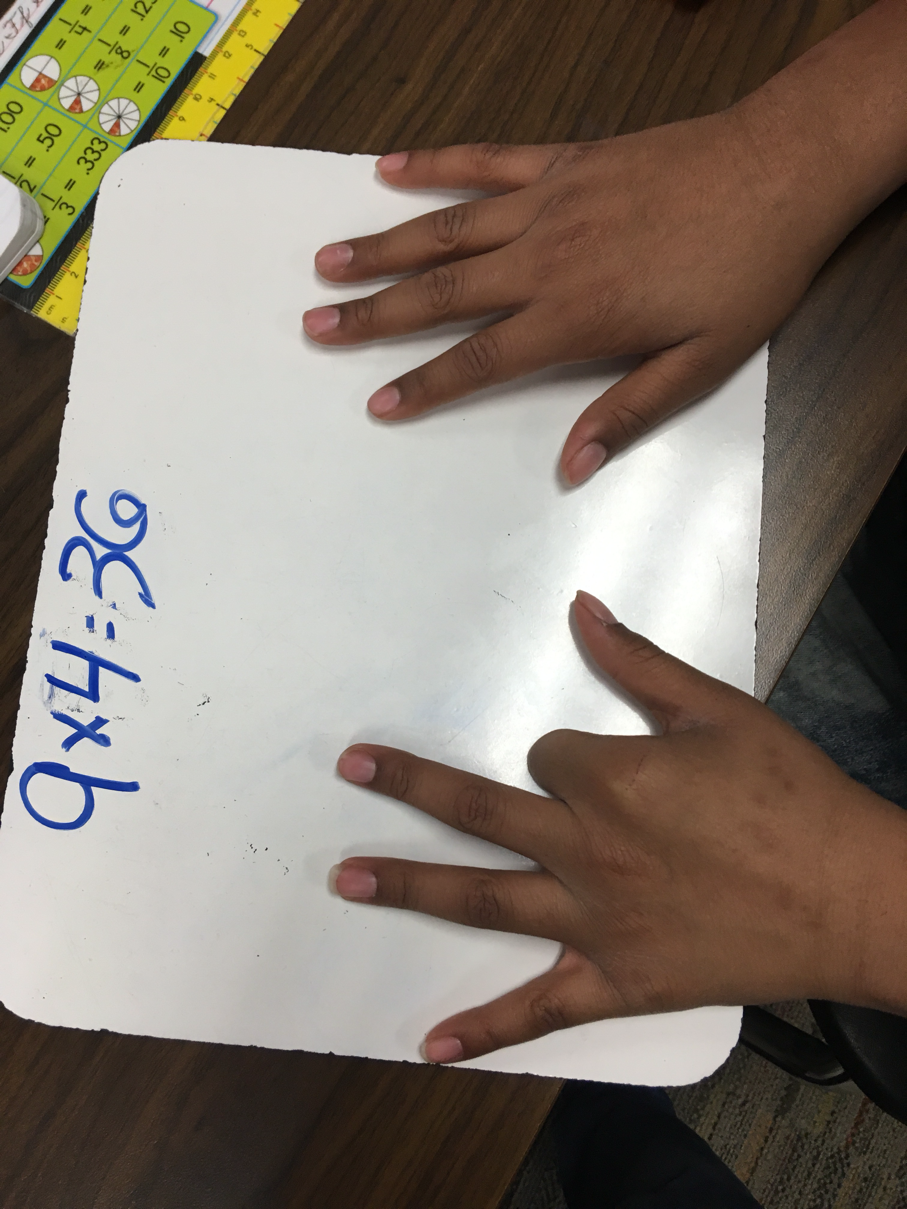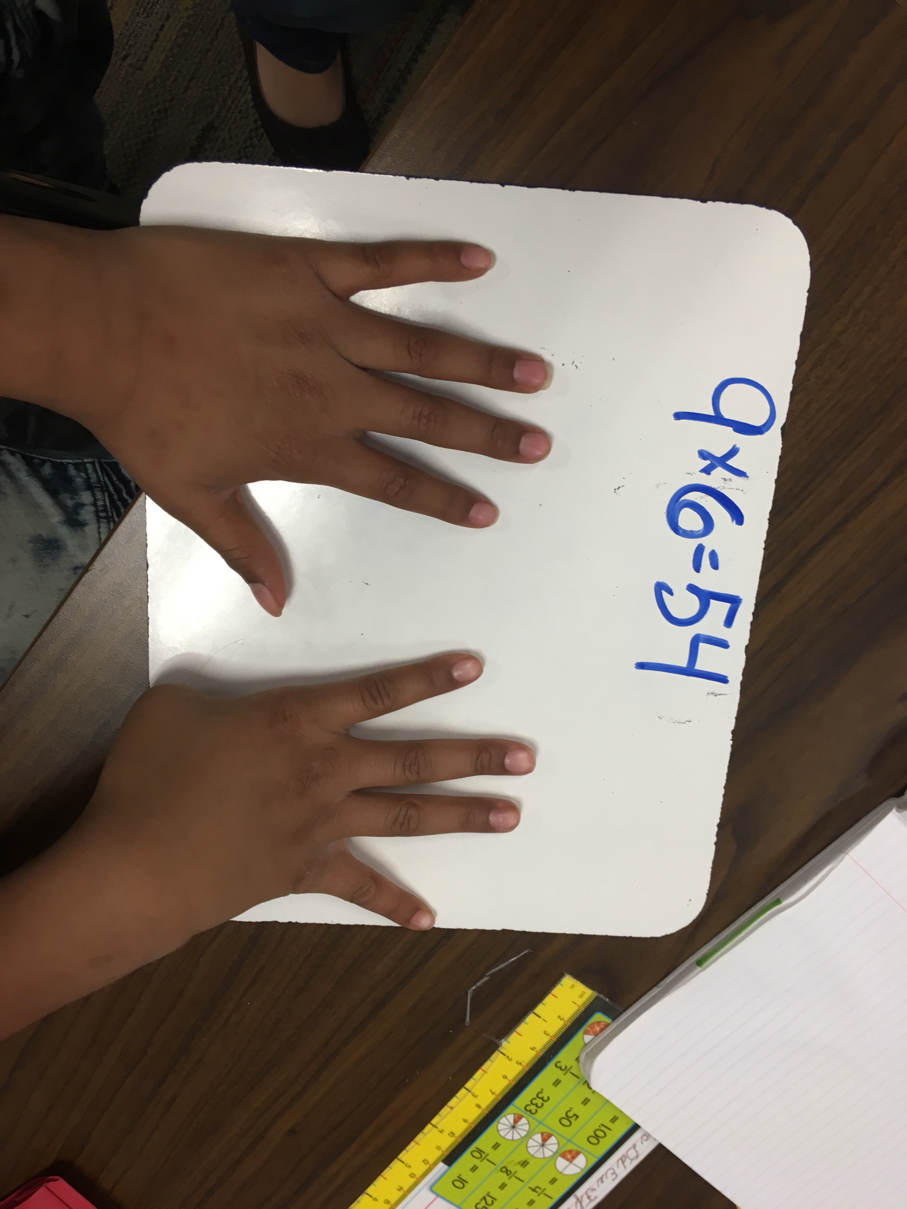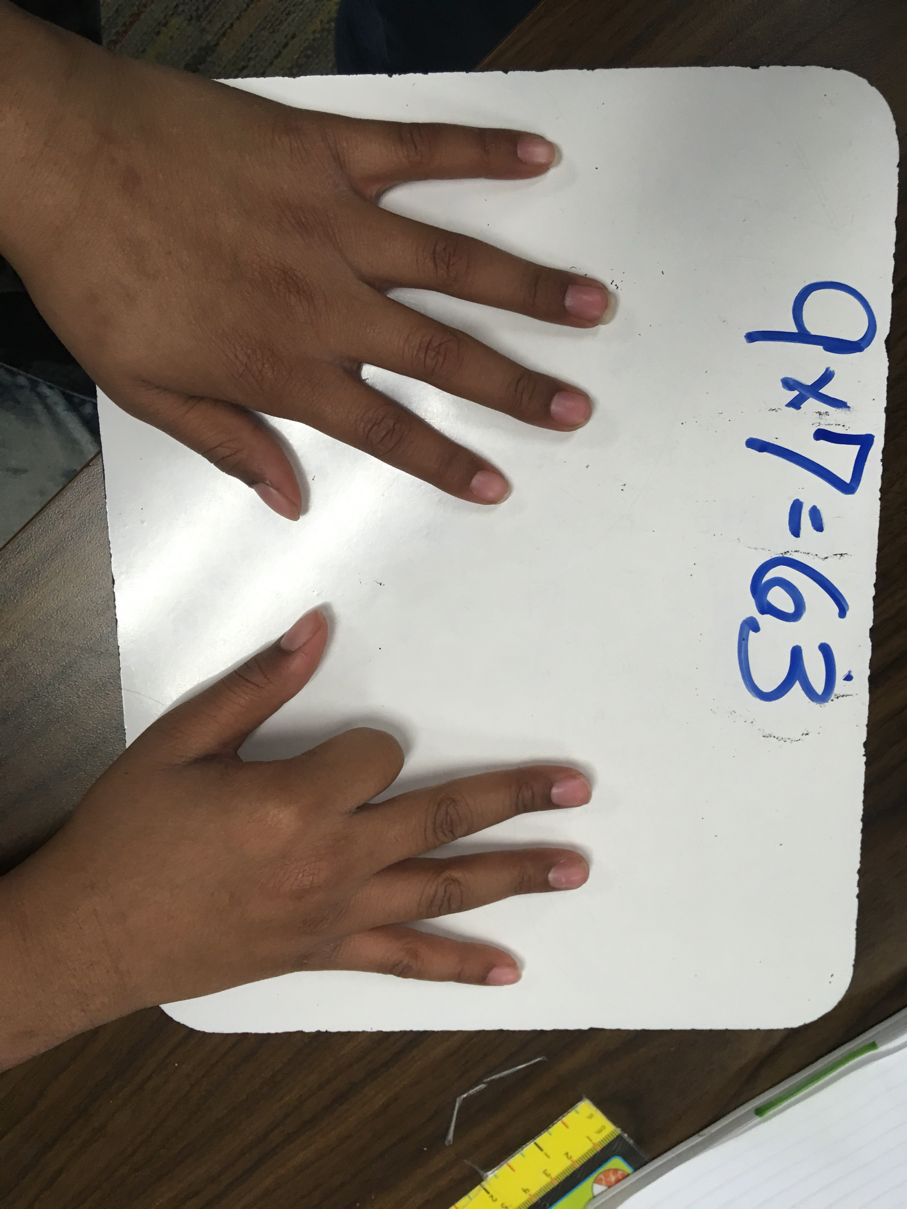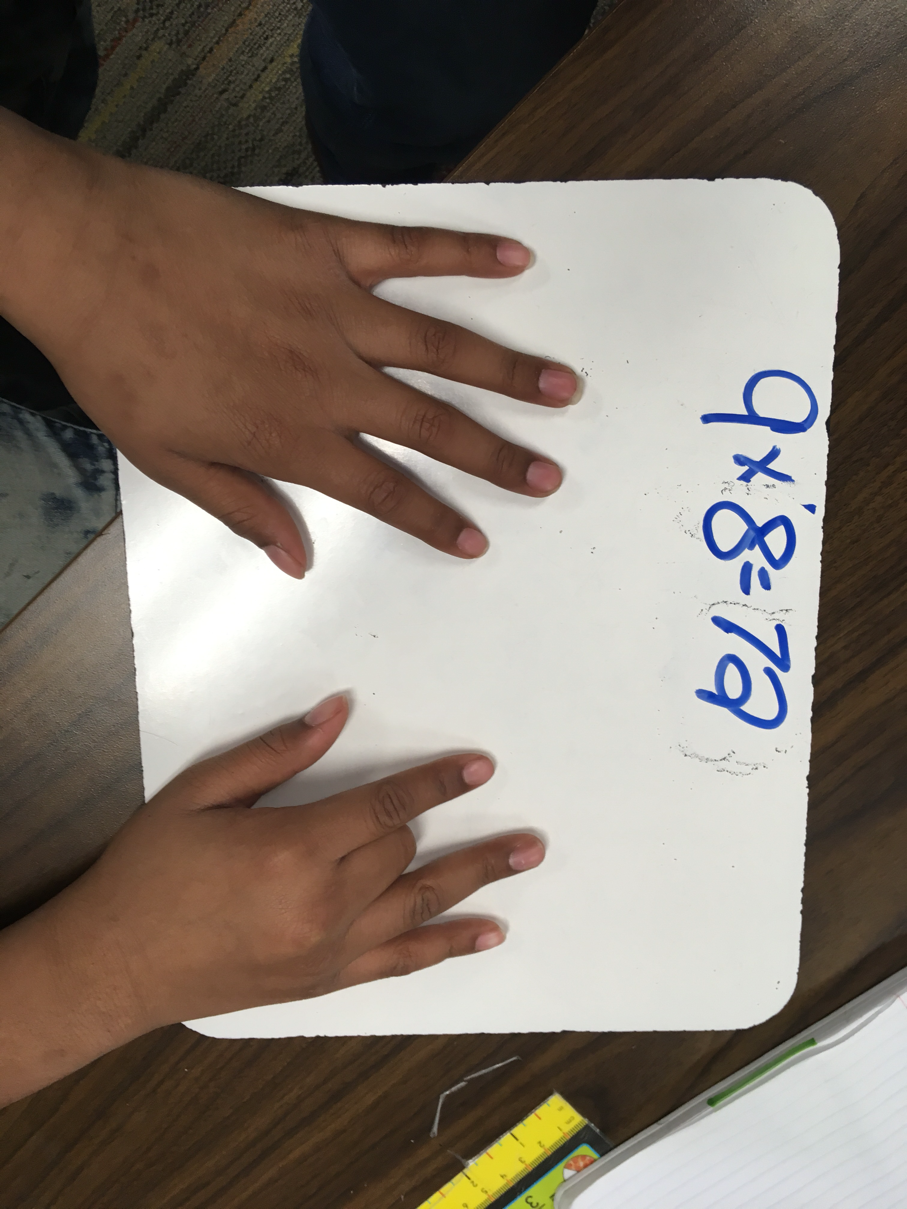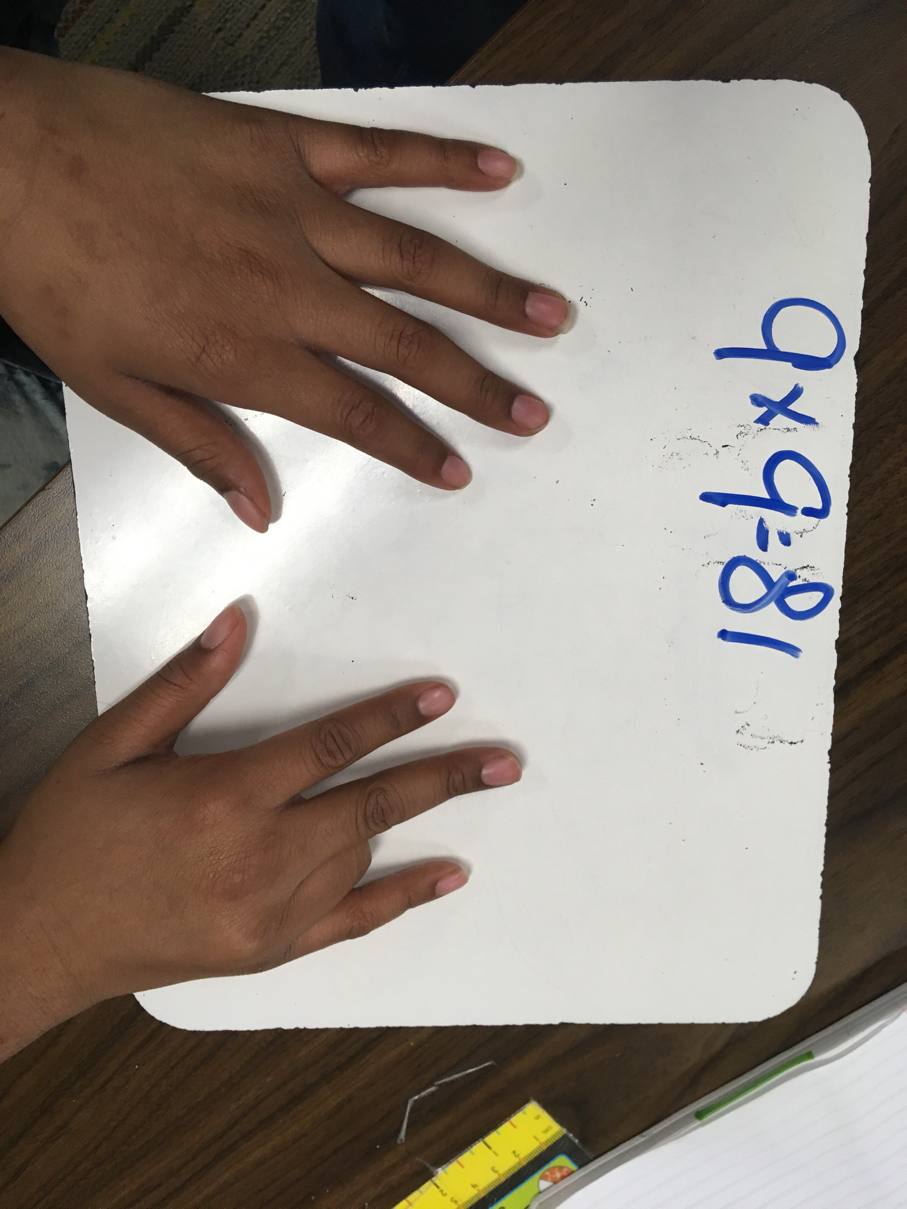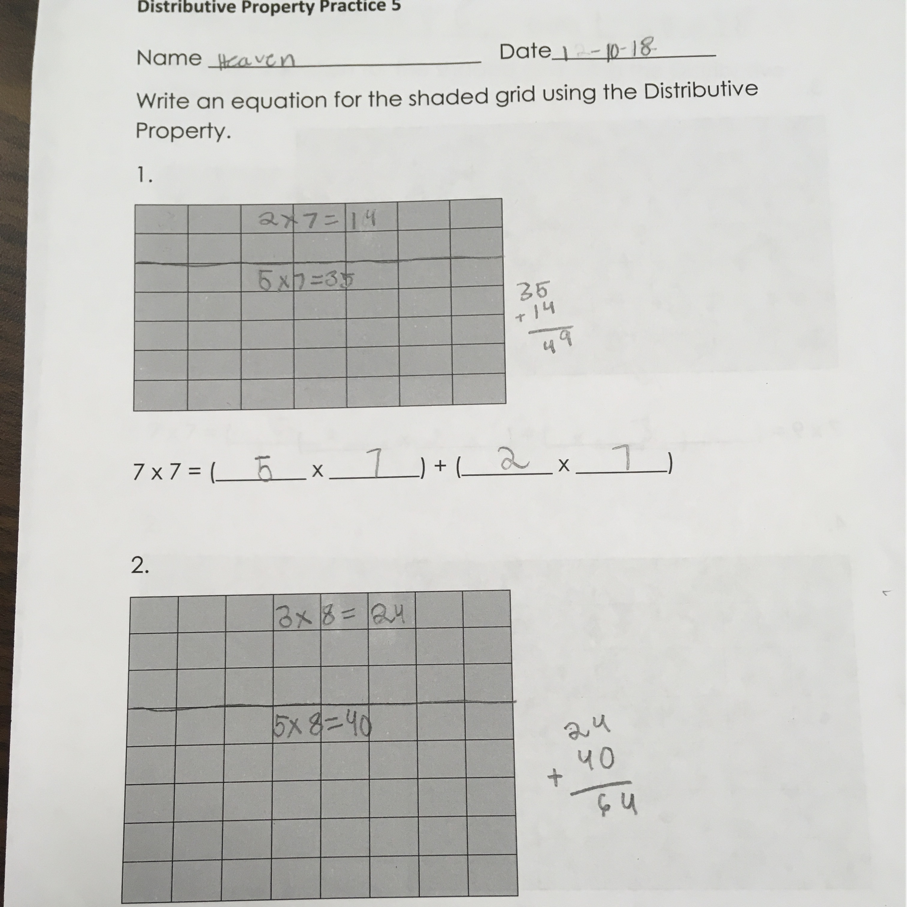Monday, January 8, 2018

Unit Five

Math
In unit five we are still working with multiplication and division.
Kiddos are expected to:
•  multiply a two digit number by a one digit number.
• represent multiplication facts using repeat addition, equations, equal-sized groups, arrays, area models, equal jumps on a number line, strip diagrams, and skip counting.
• have their facts up to 10 x 10 memorized.
• determine if a number is even or odd.
• represent relationships using an input output table.

Science
In unit five we're moving to mixtures and soil.
They will explore that mixtures are created when two materials are combined.
They'll learn that soil is formed by weathering of rocks and decomposition of plants and animals remains.

Thursday, December 21, 2017

Holiday Party